# Selina Solutions Concise Mathematics Class 6 Chapter 11 Ratio

Selina Solutions Concise Mathematics Class 6 Chapter 11 Ratio gives a strong knowledge about basic concepts, so that students can build a better hold on the subject. By practising Selina Solutions chapter wise problems precisely, students score good marks in final examination. The expert faculty at BYJU’S have curated the solutions based on students’ understanding abilities. Solutions PDF are available in a simple and lucid manner. Selina Solutions Concise Mathematics Class 6 Chapter 11 Ratio PDF links are provided, for free download

Chapter 11 covers basic concepts such as Ratio and Proportion. Students get to know various operations of Ratio by going through Selina Solutions in detail. Also, students obtain a fundamental idea of concepts covered here. For a better understanding of the concepts, solutions are created in step wise manner with neat explanations

## Selina Solutions Concise Mathematics Class 6 Chapter 11: Ratio Download PDF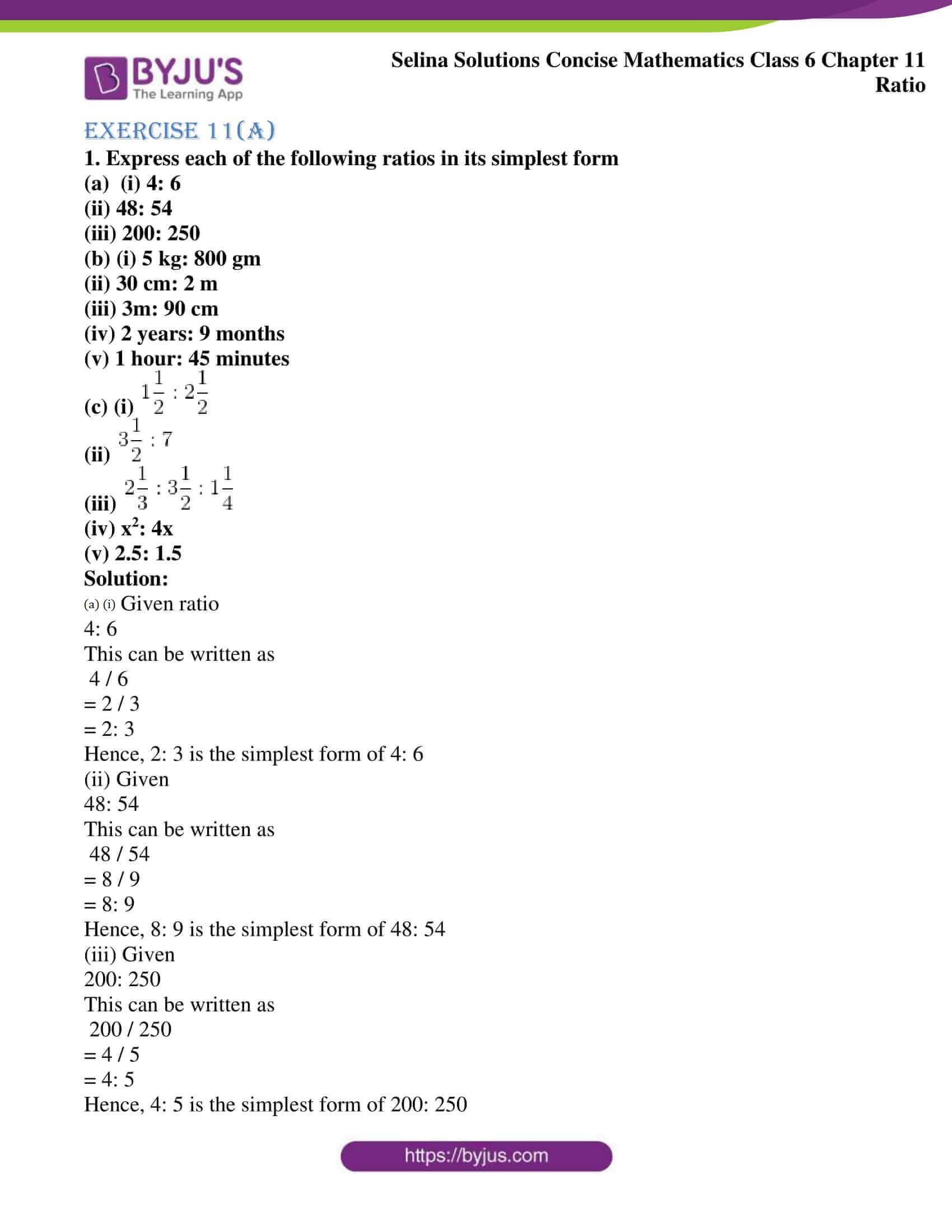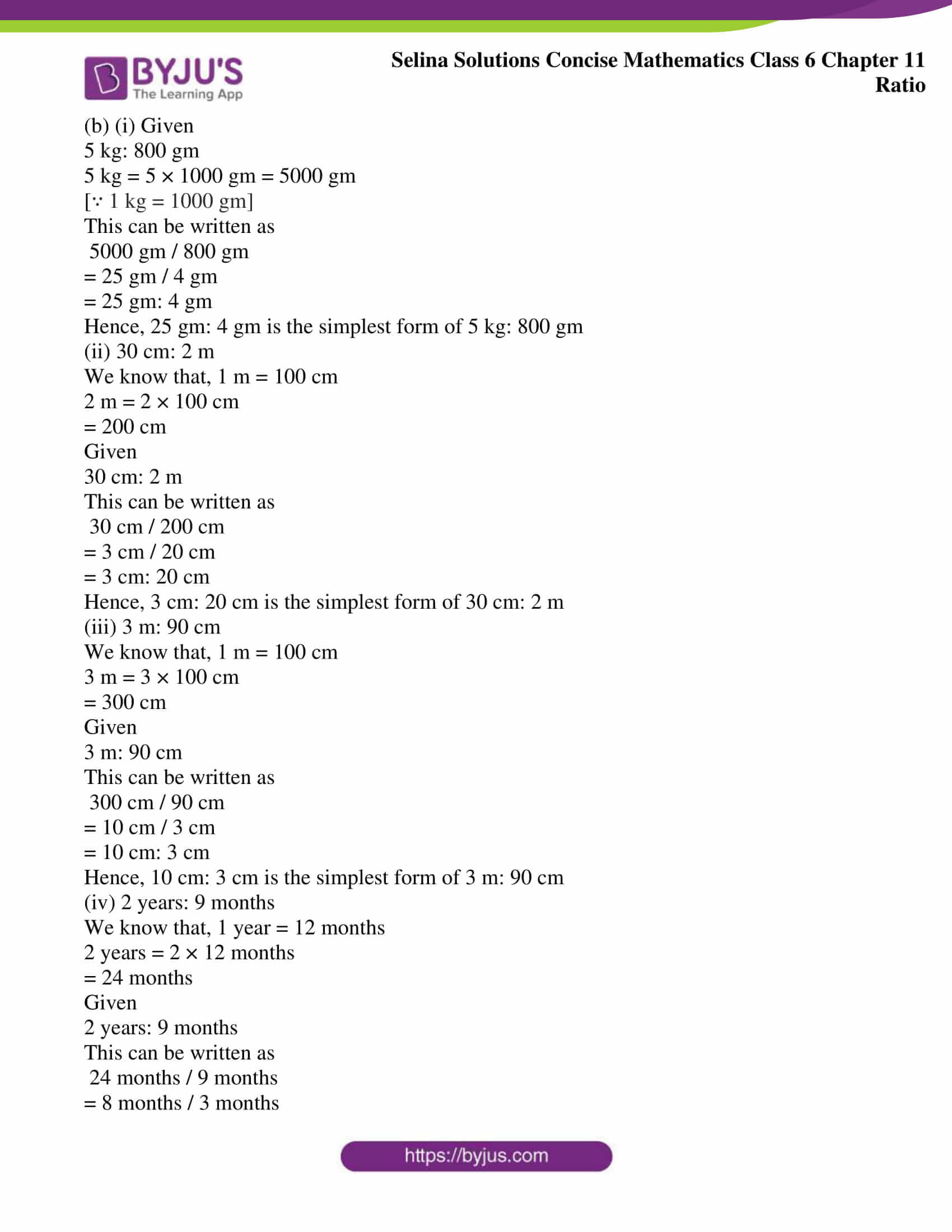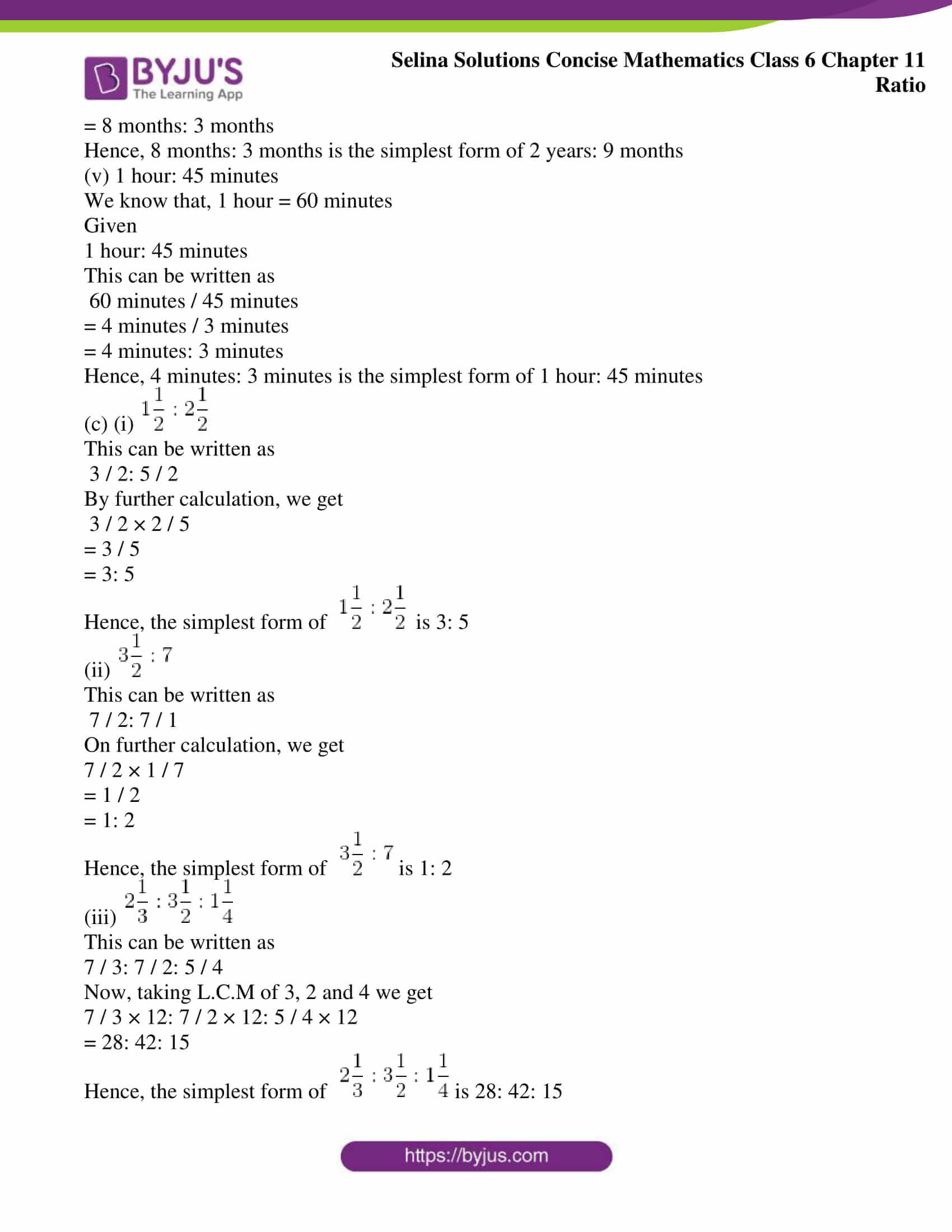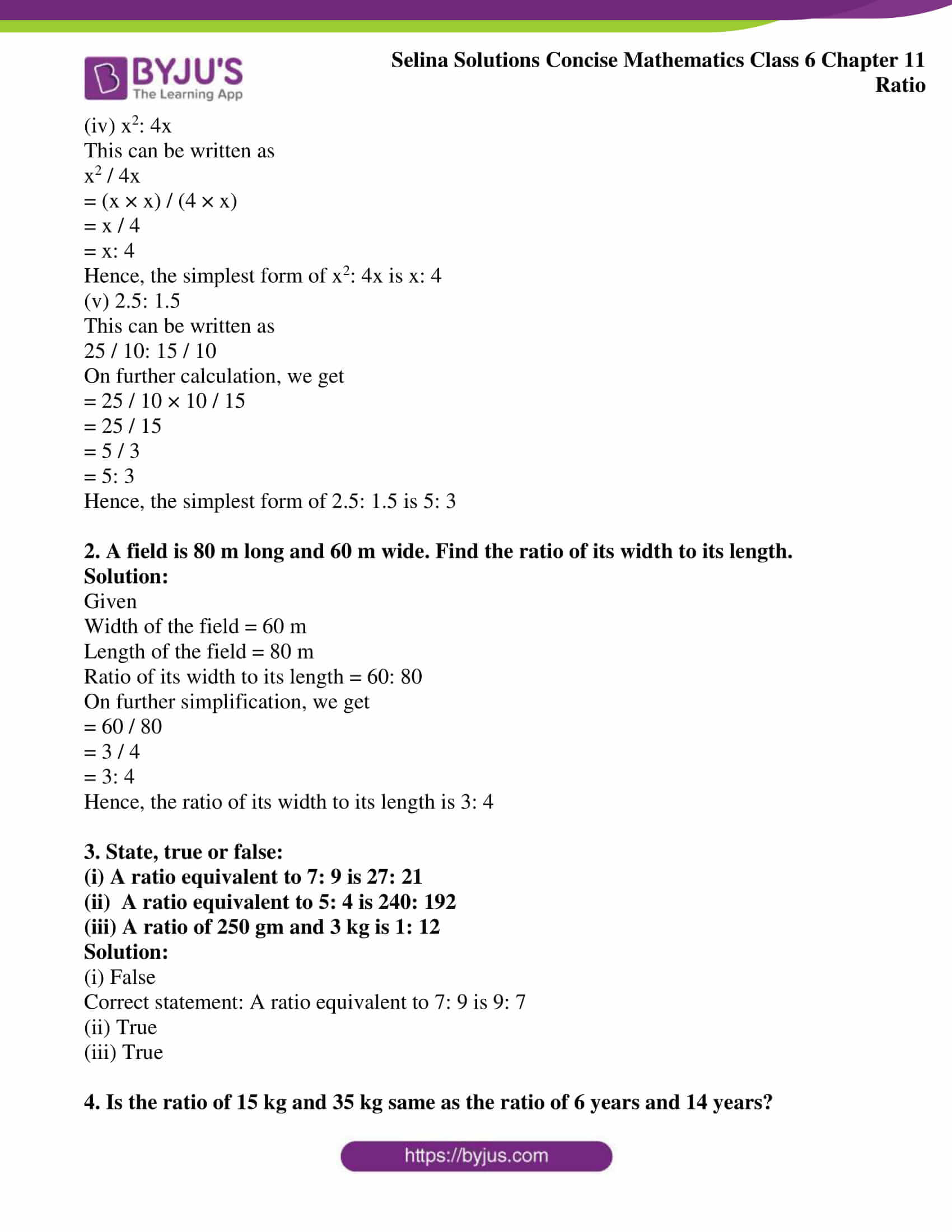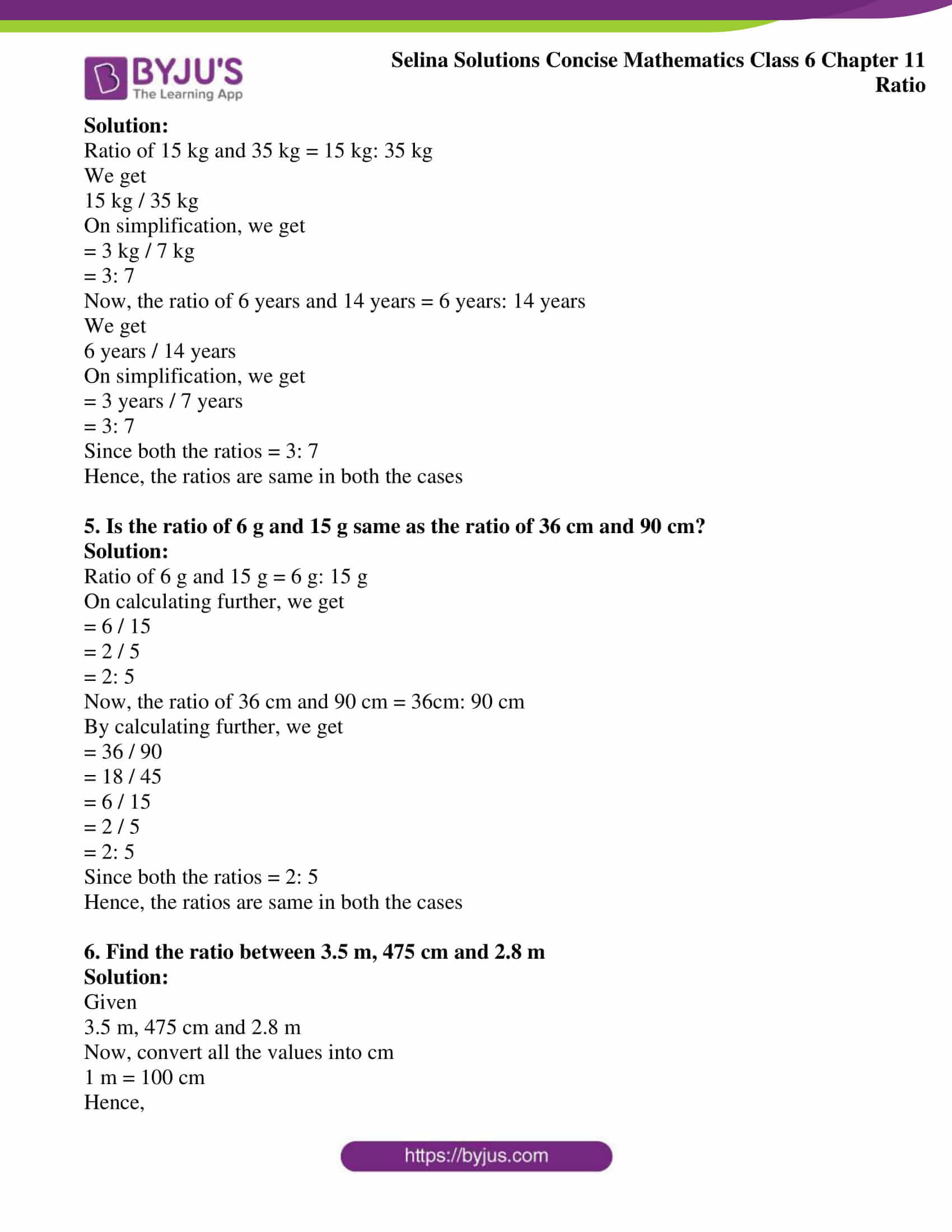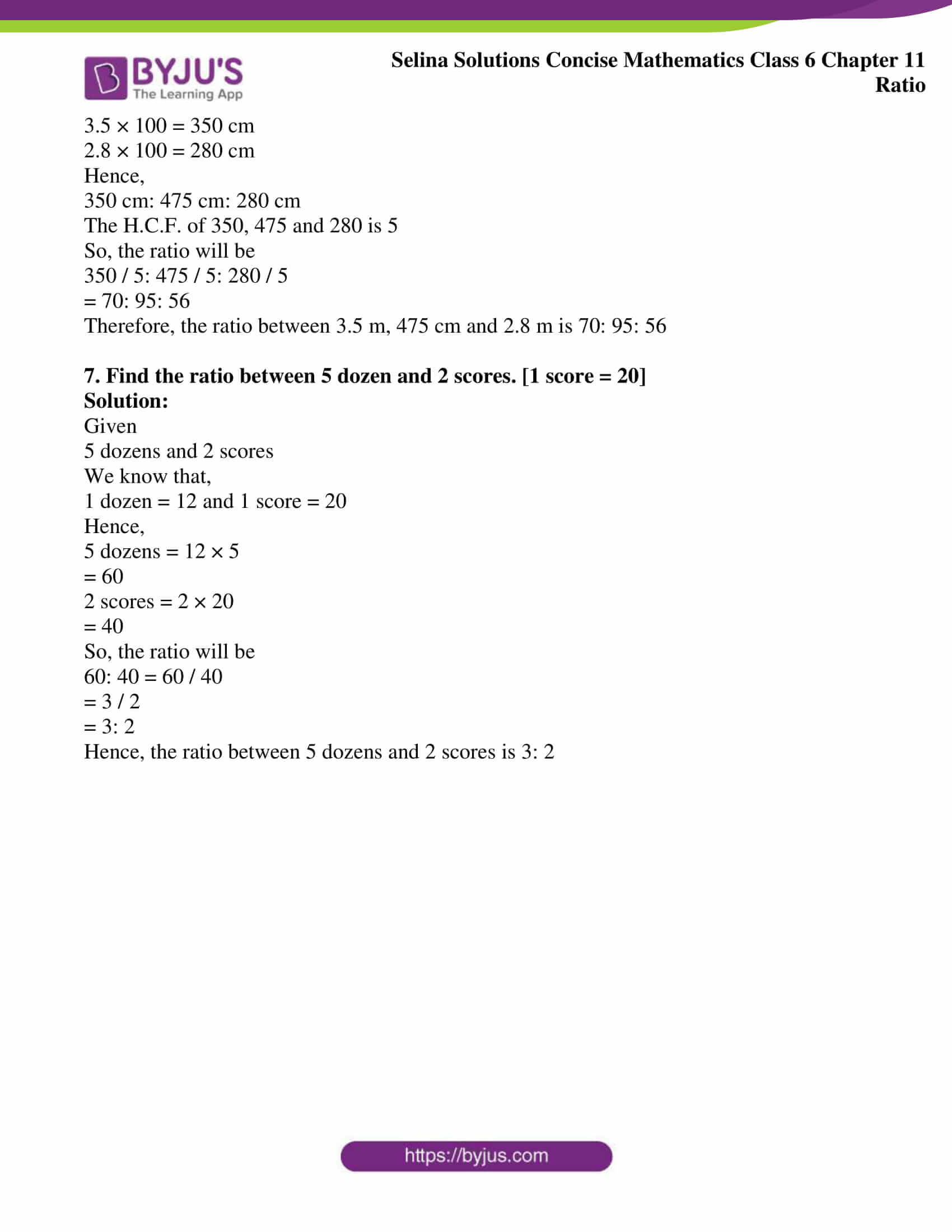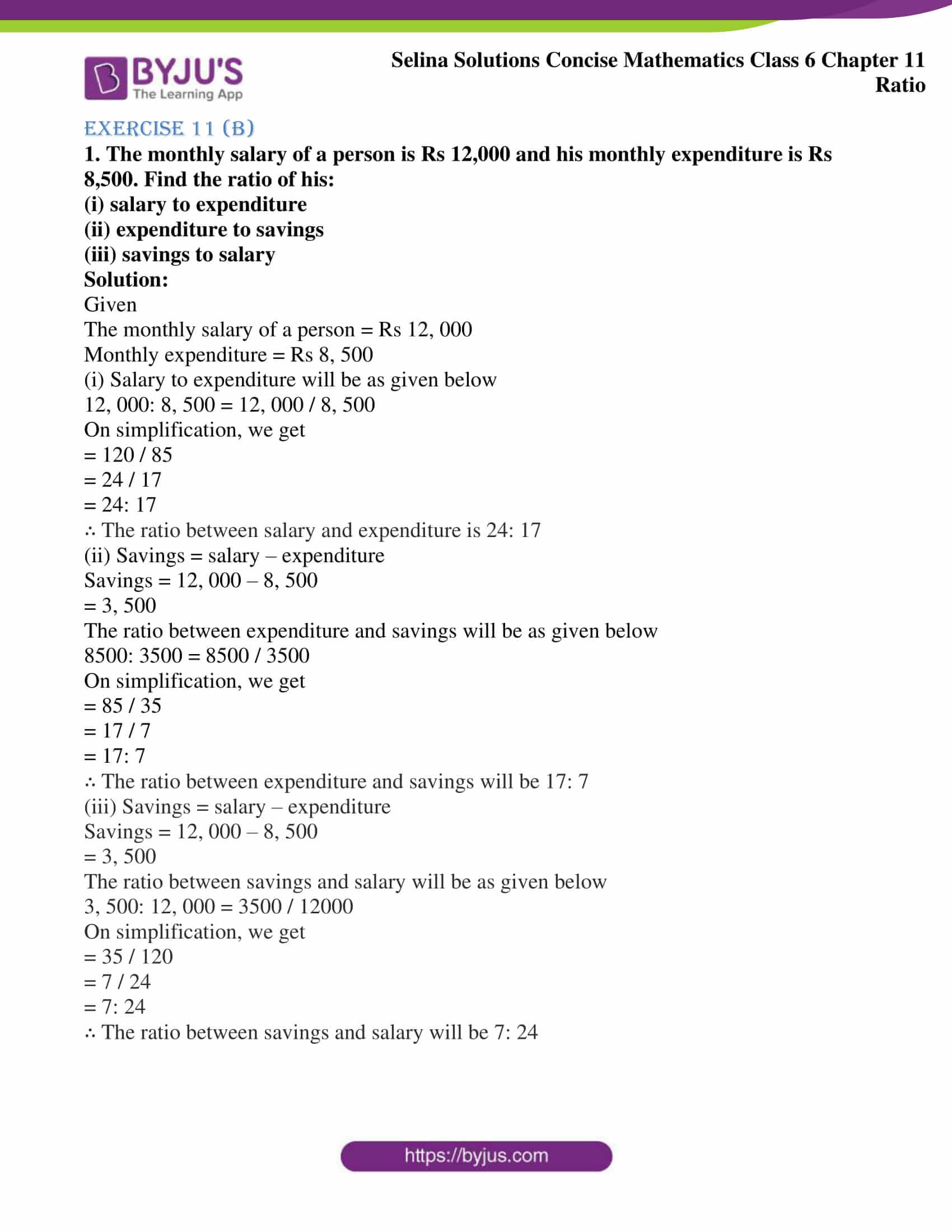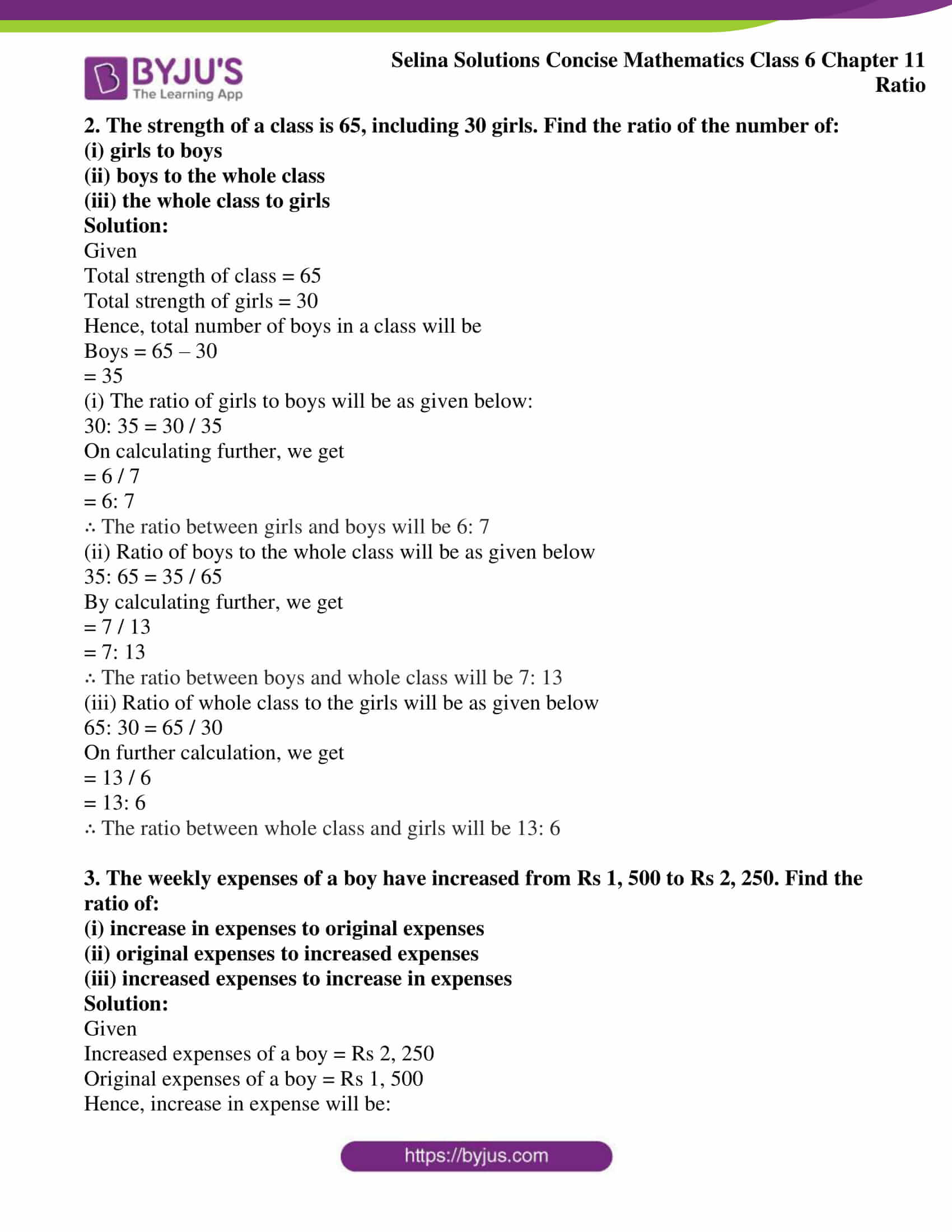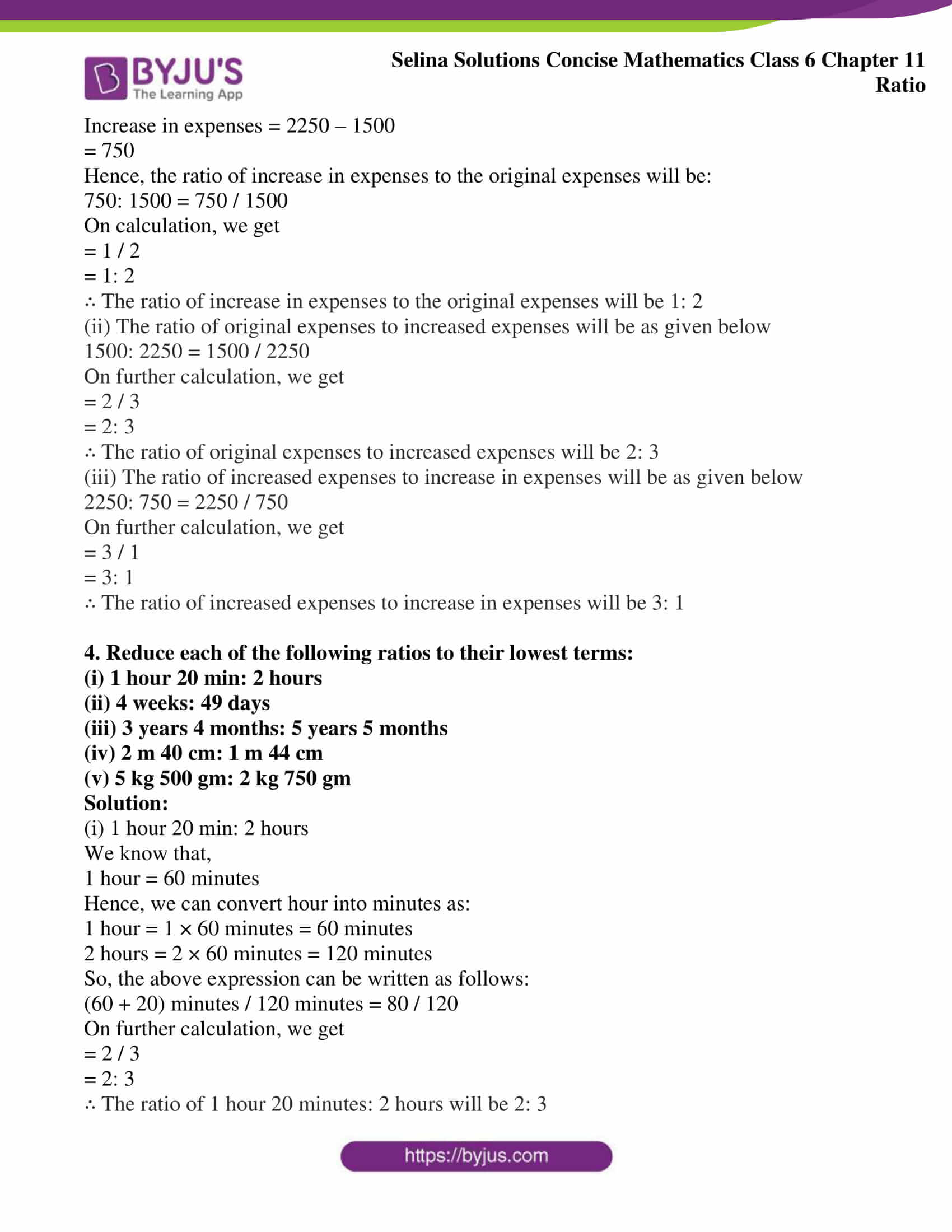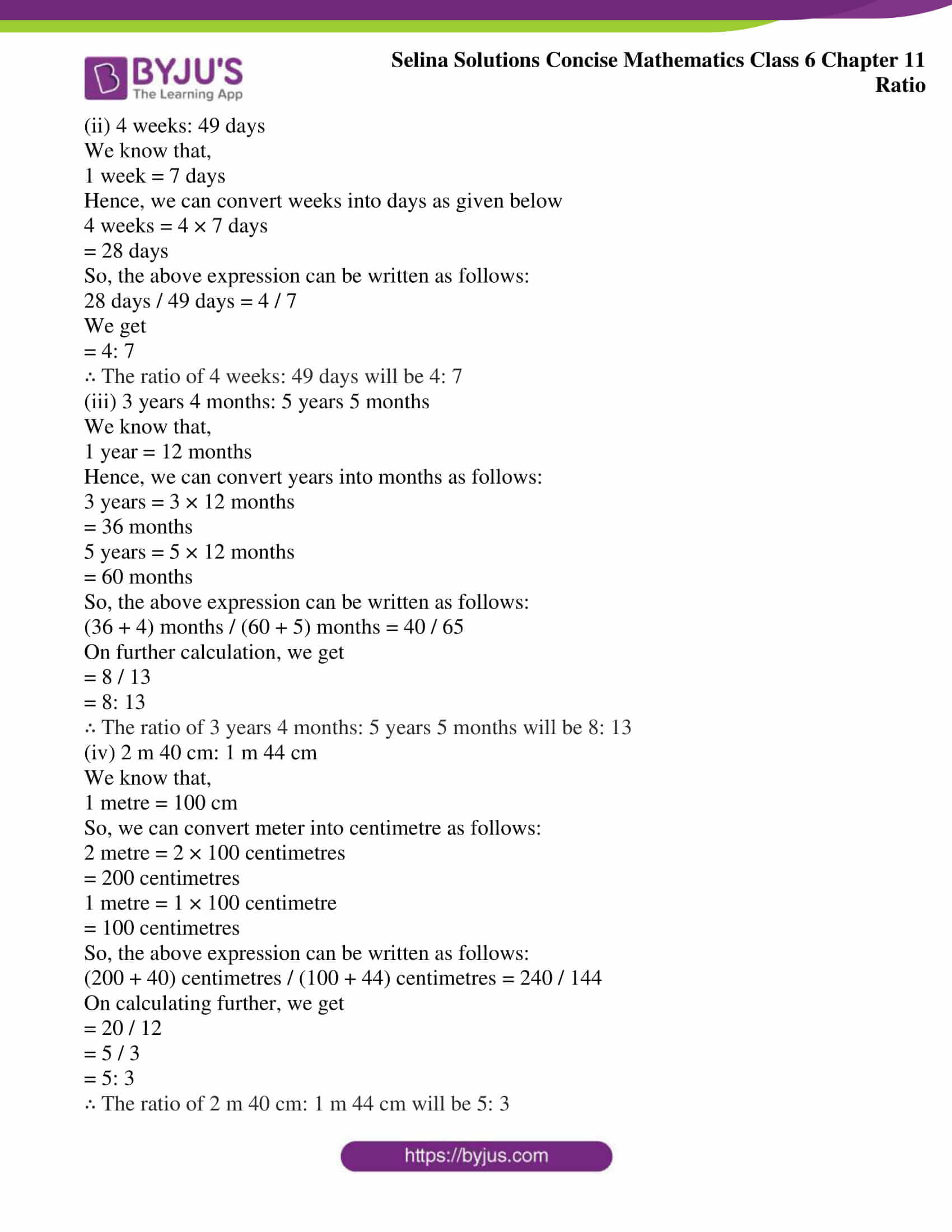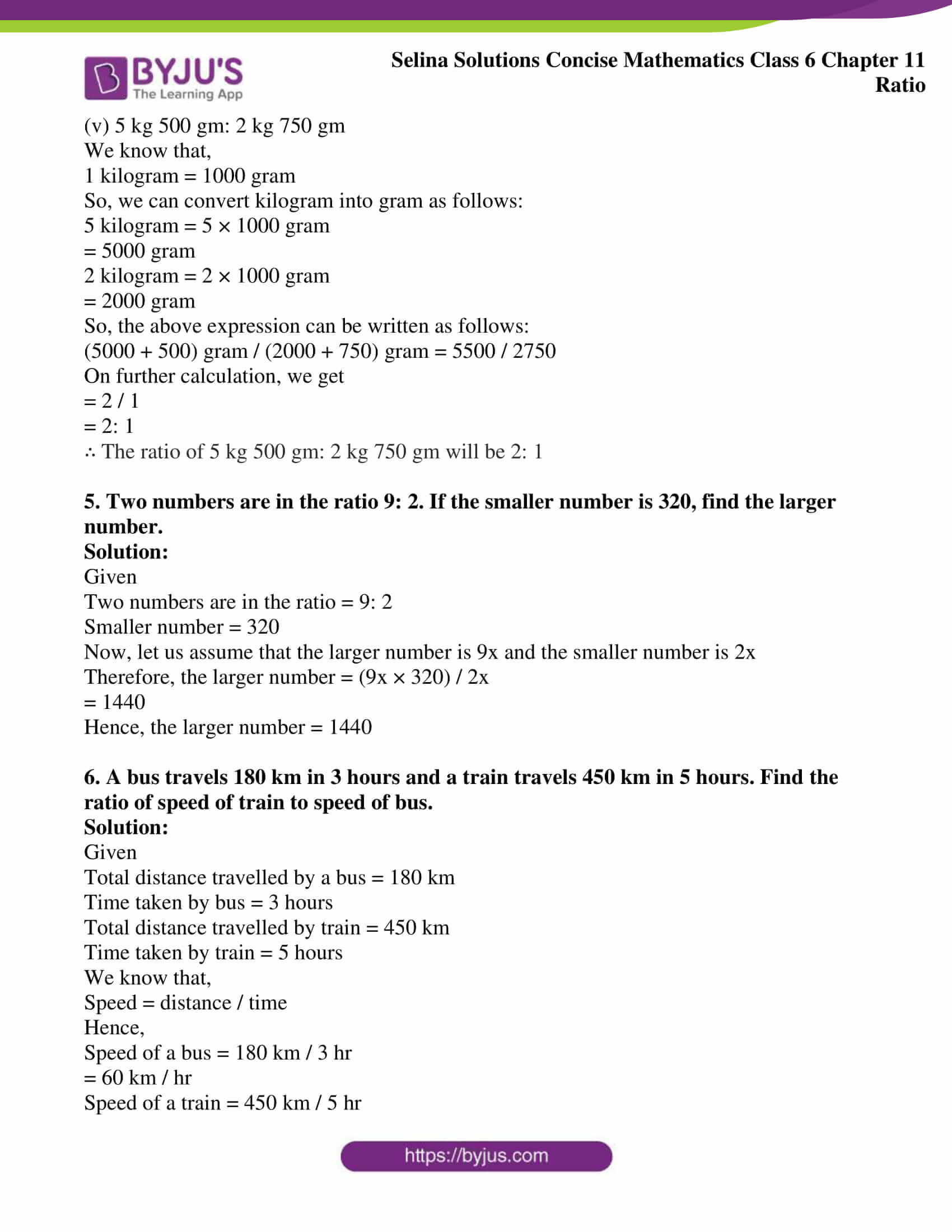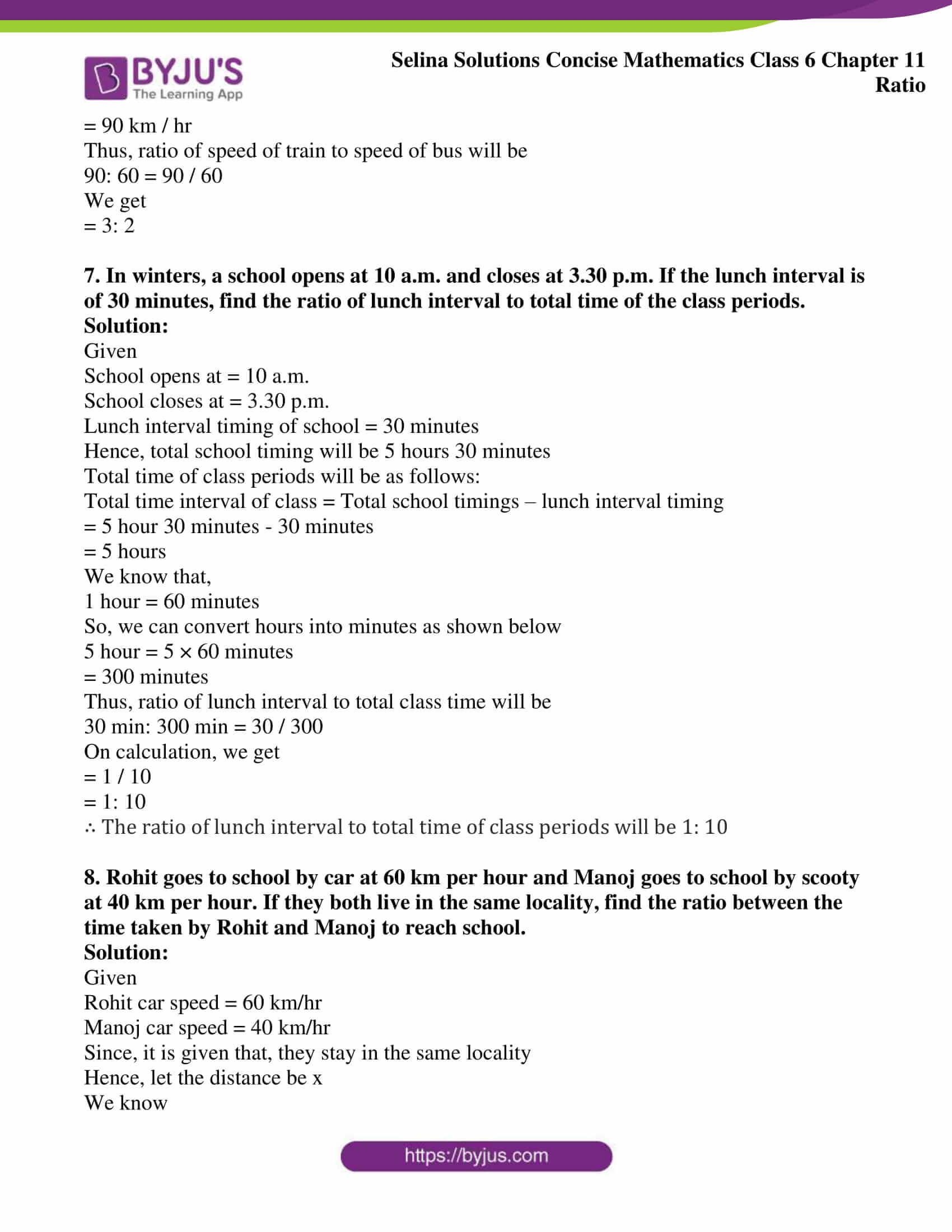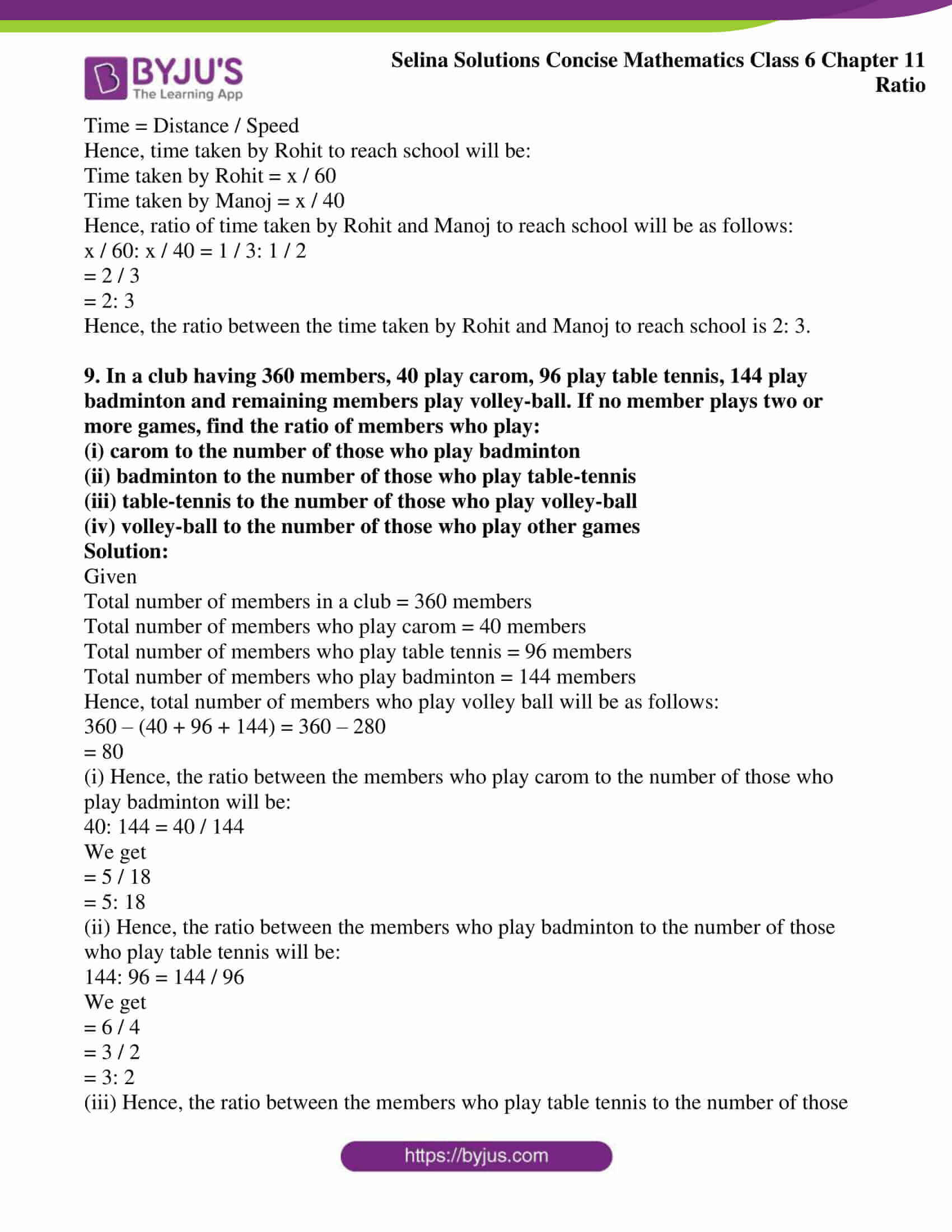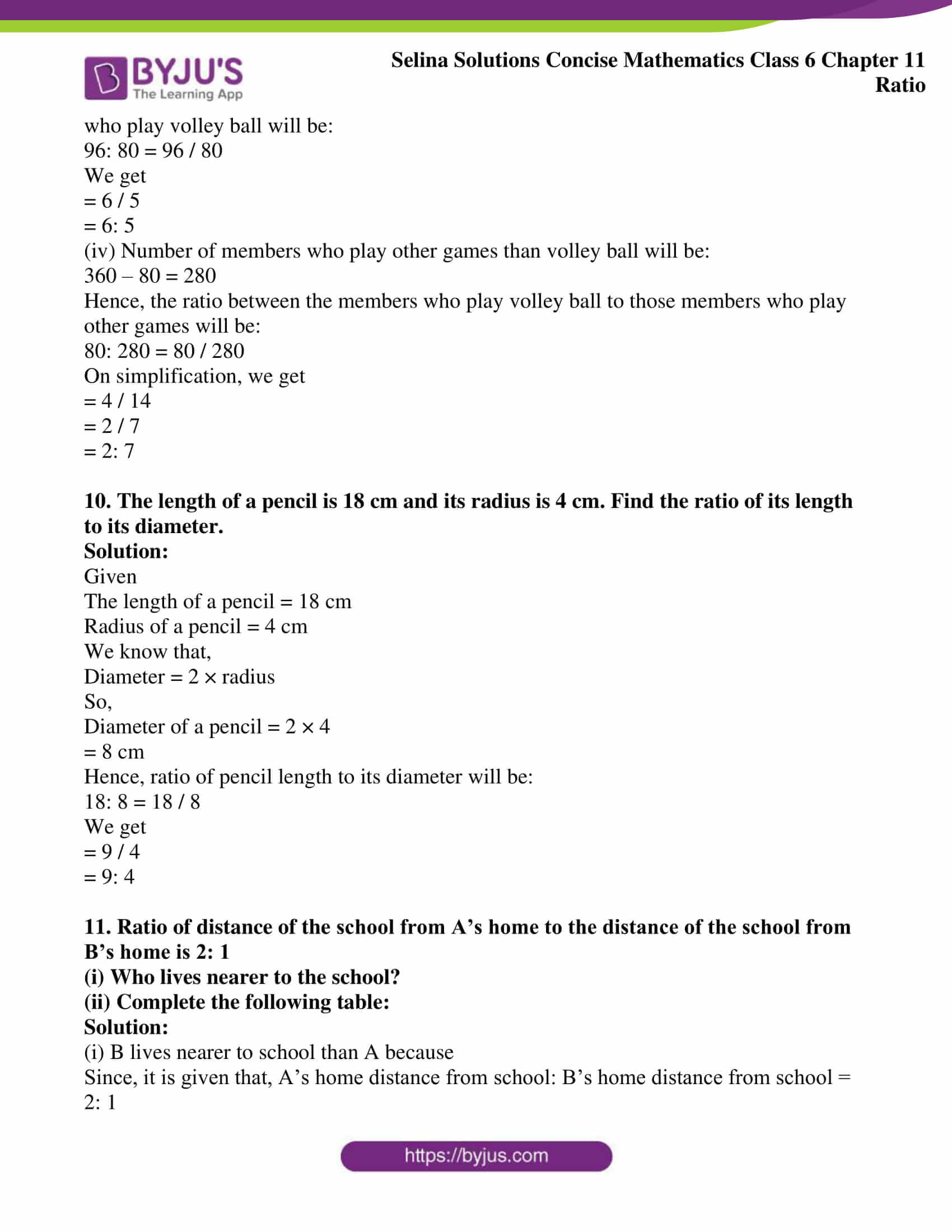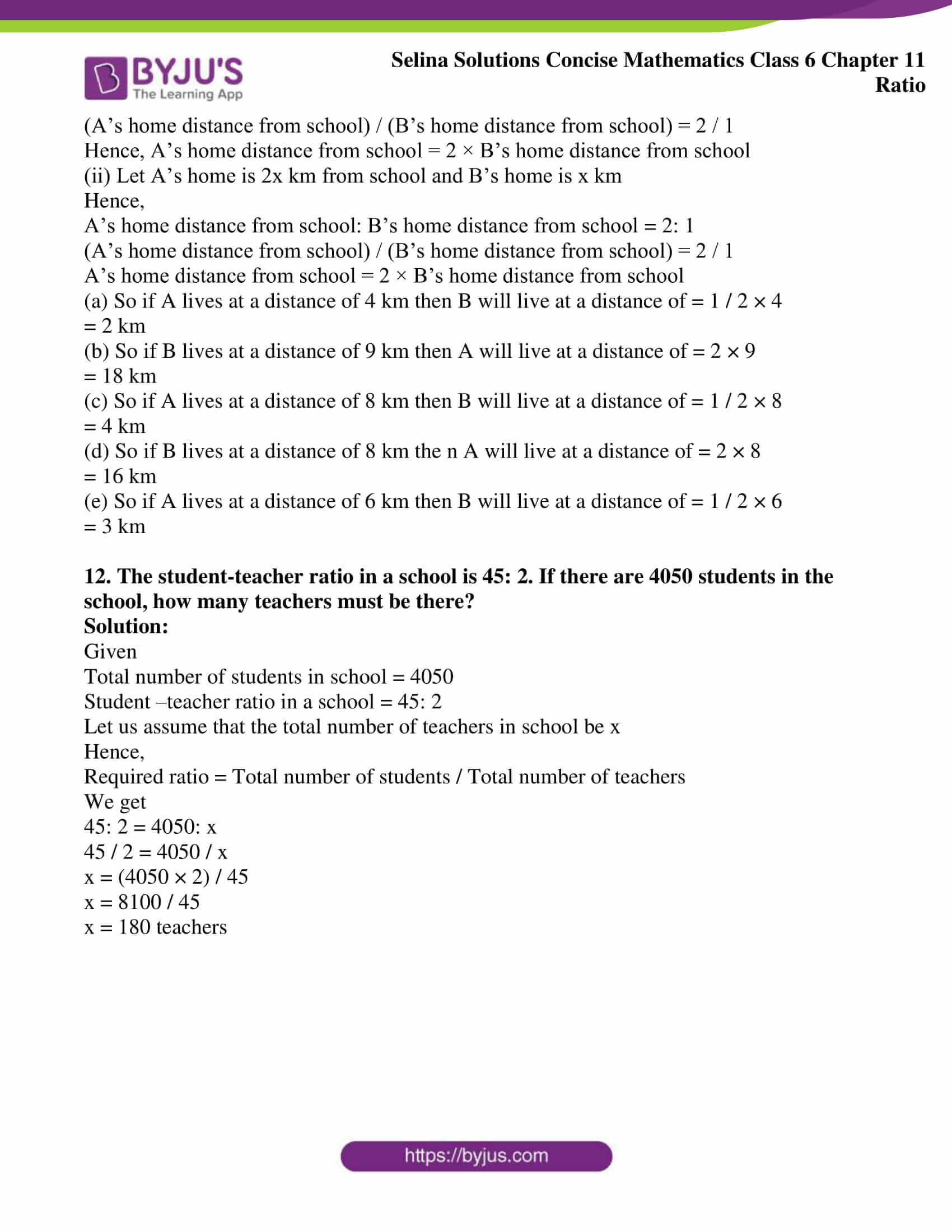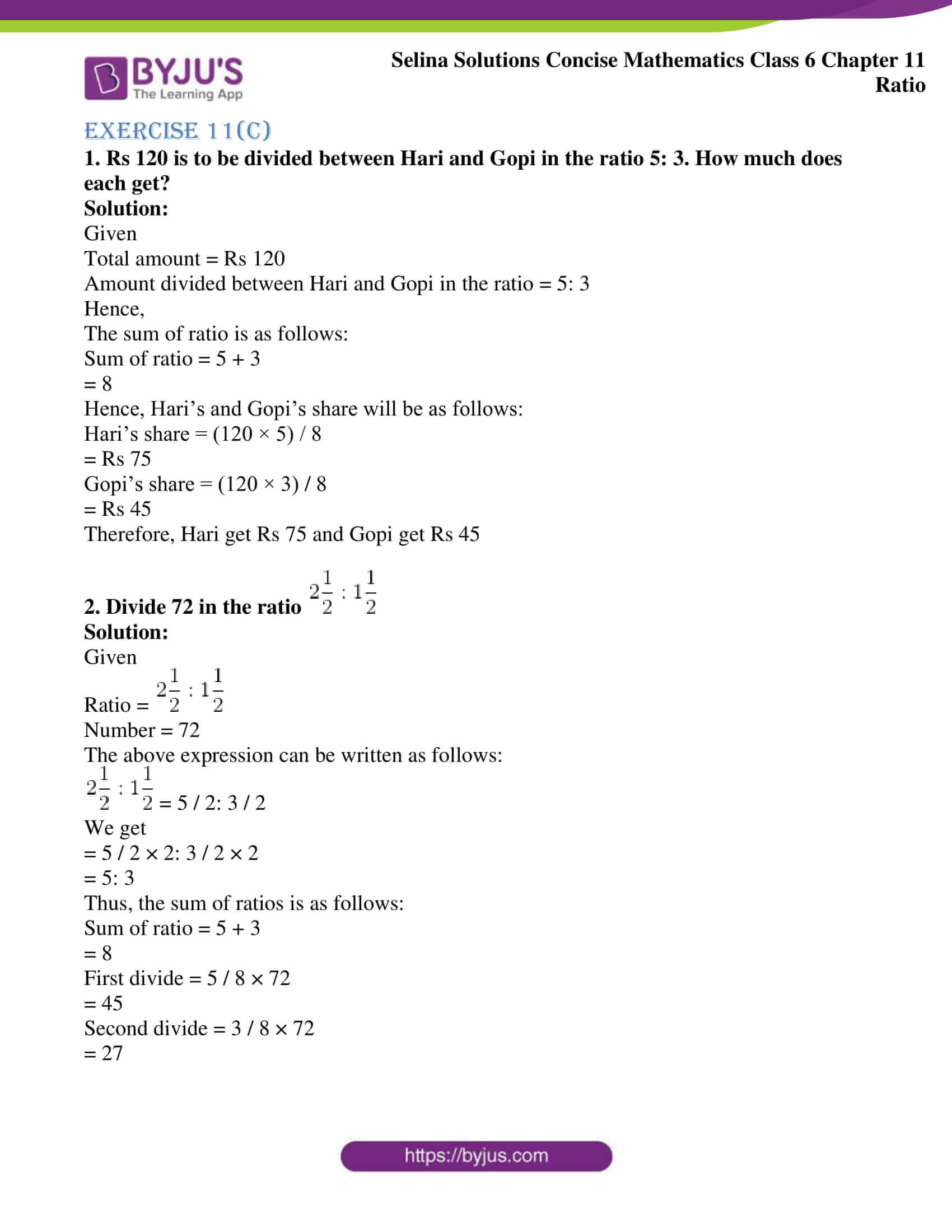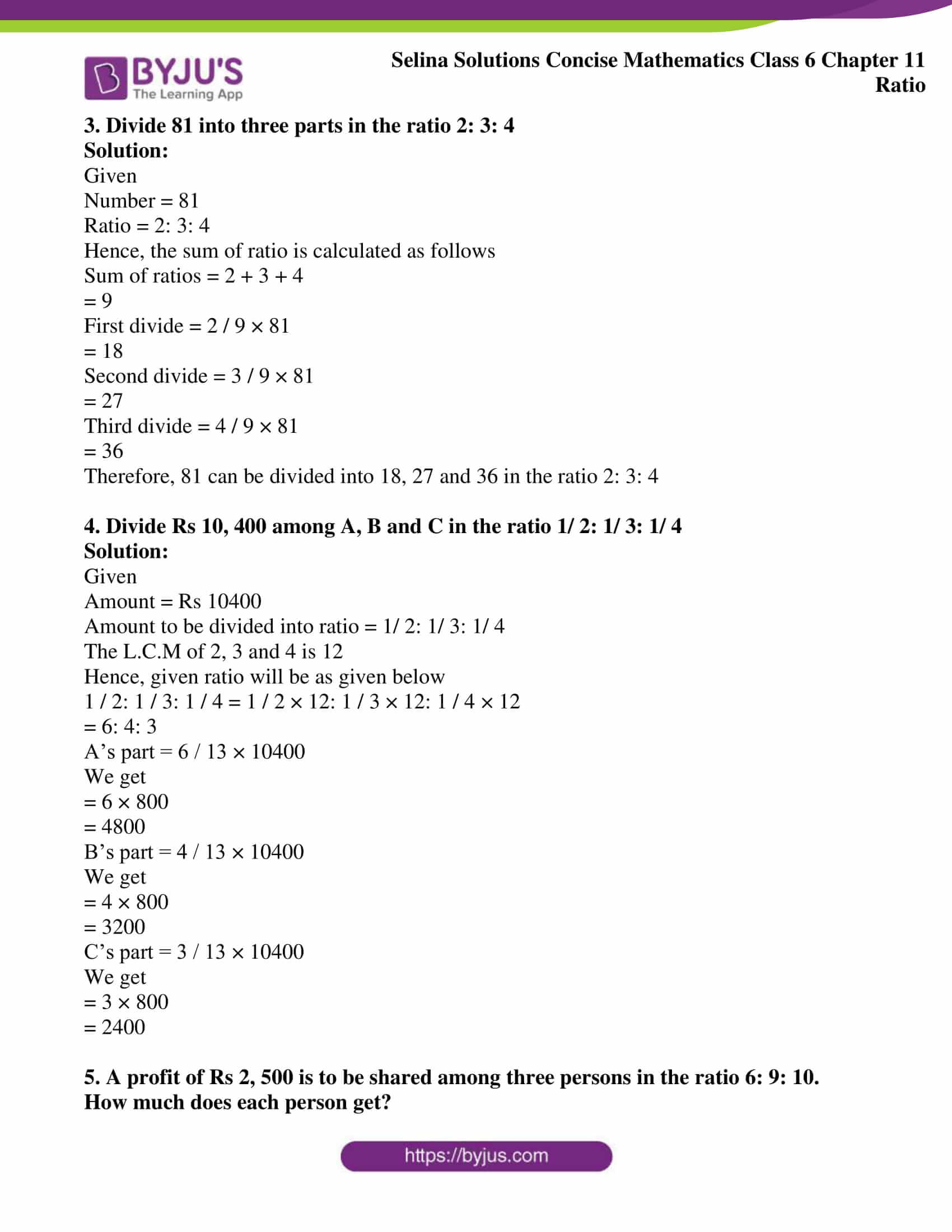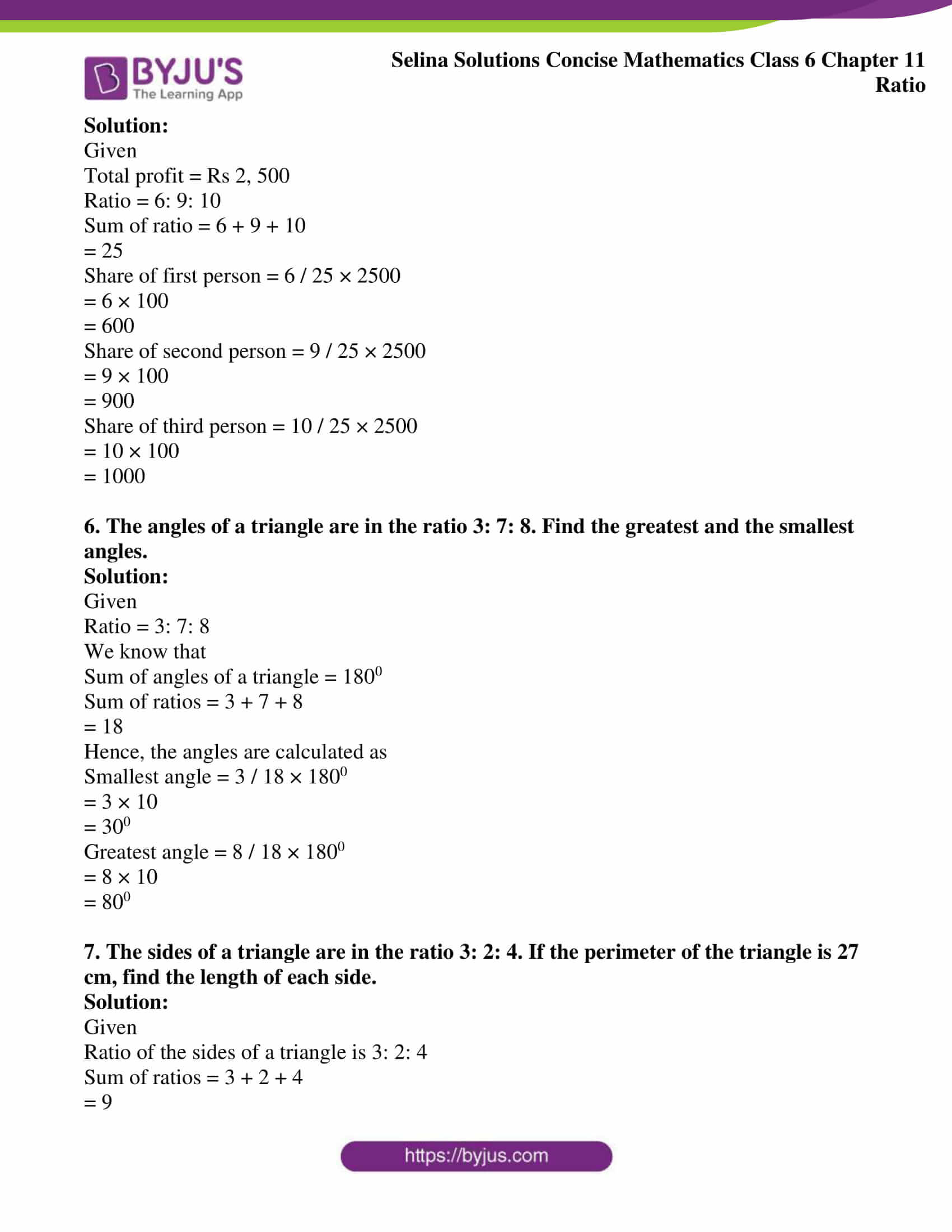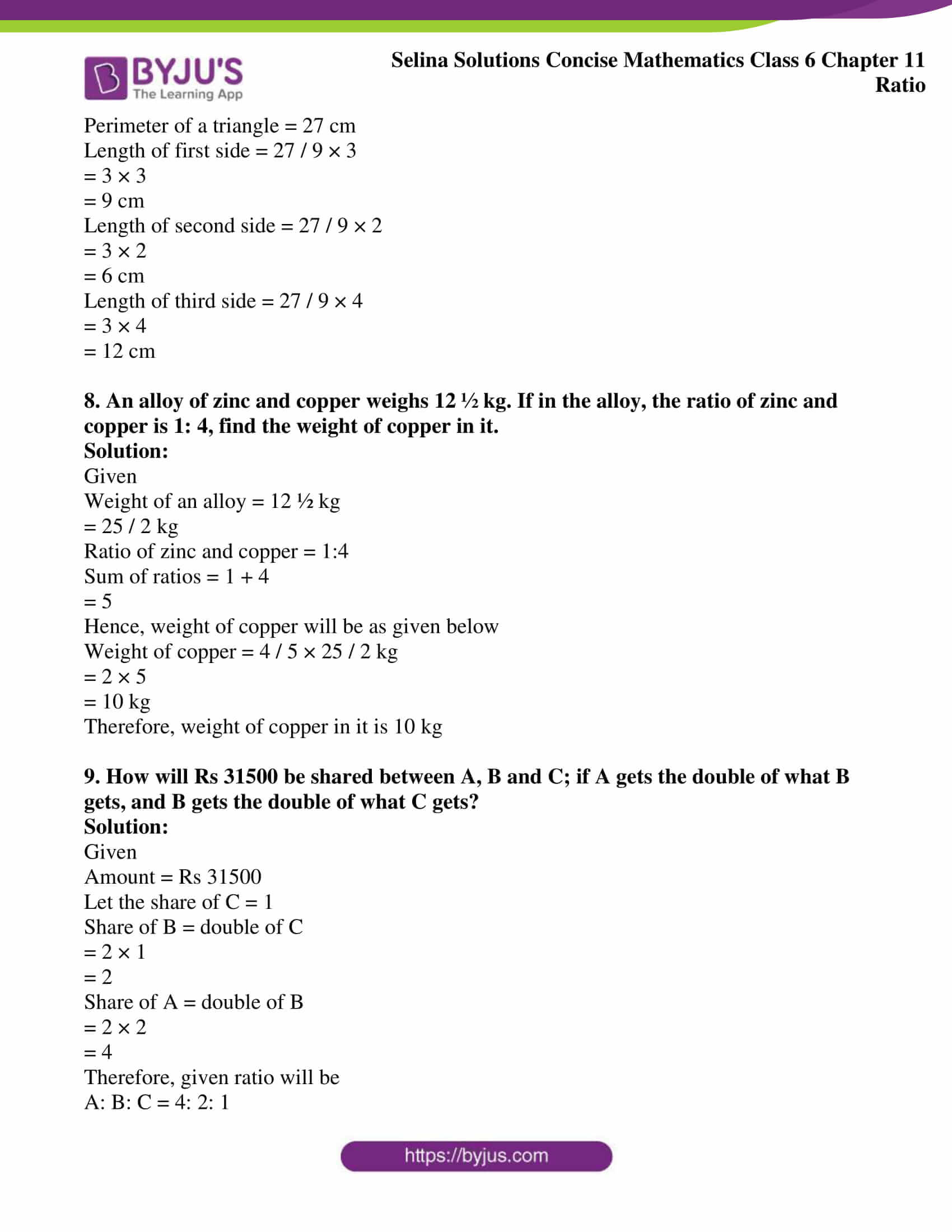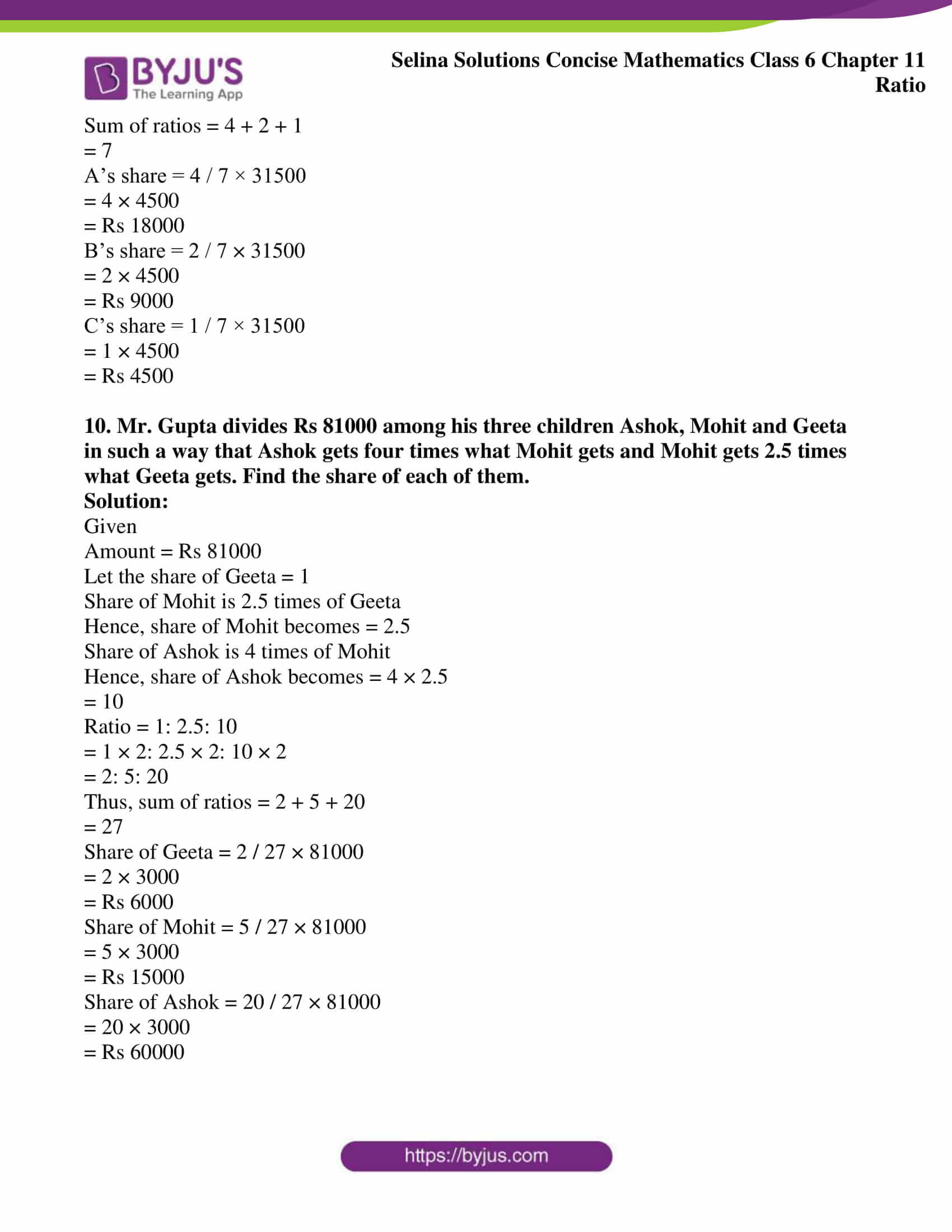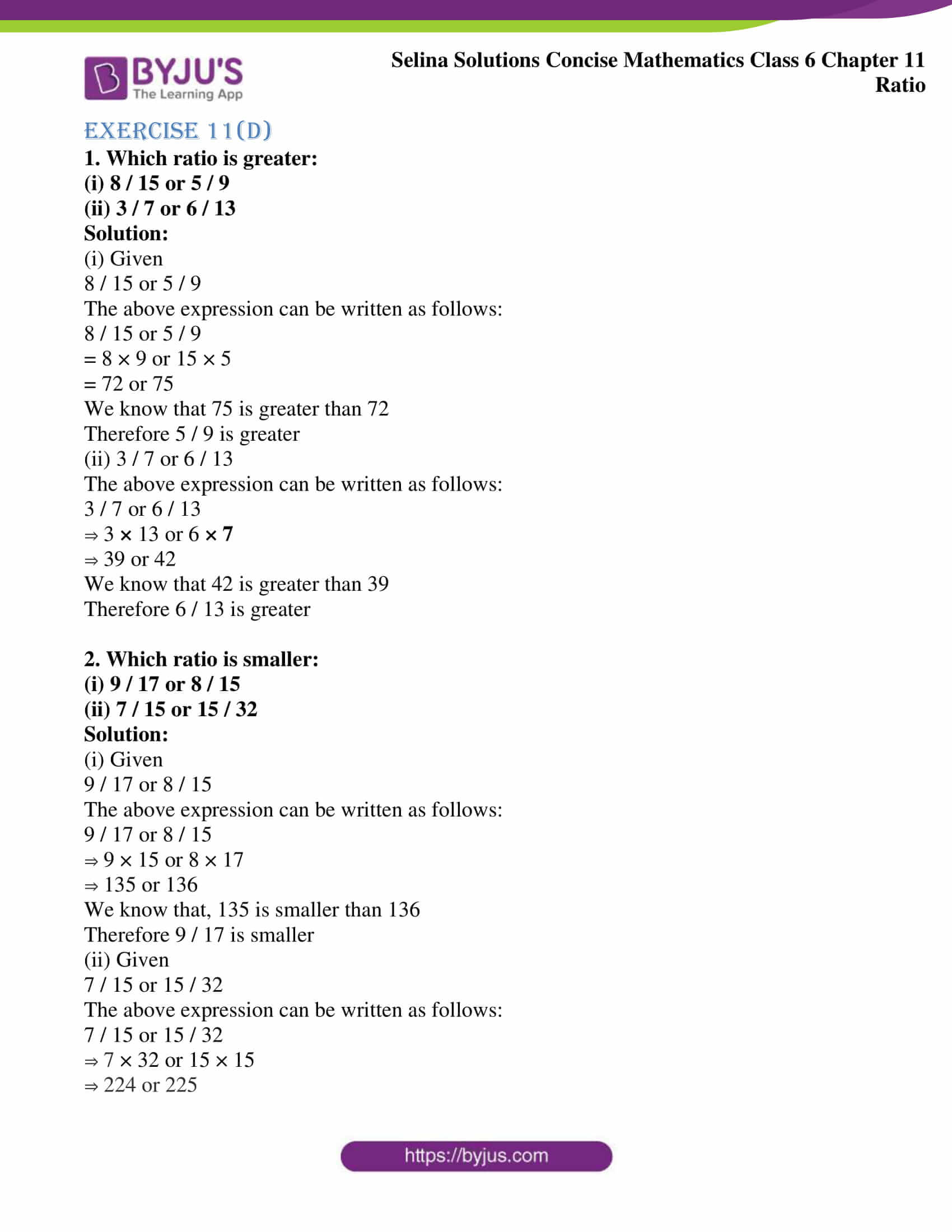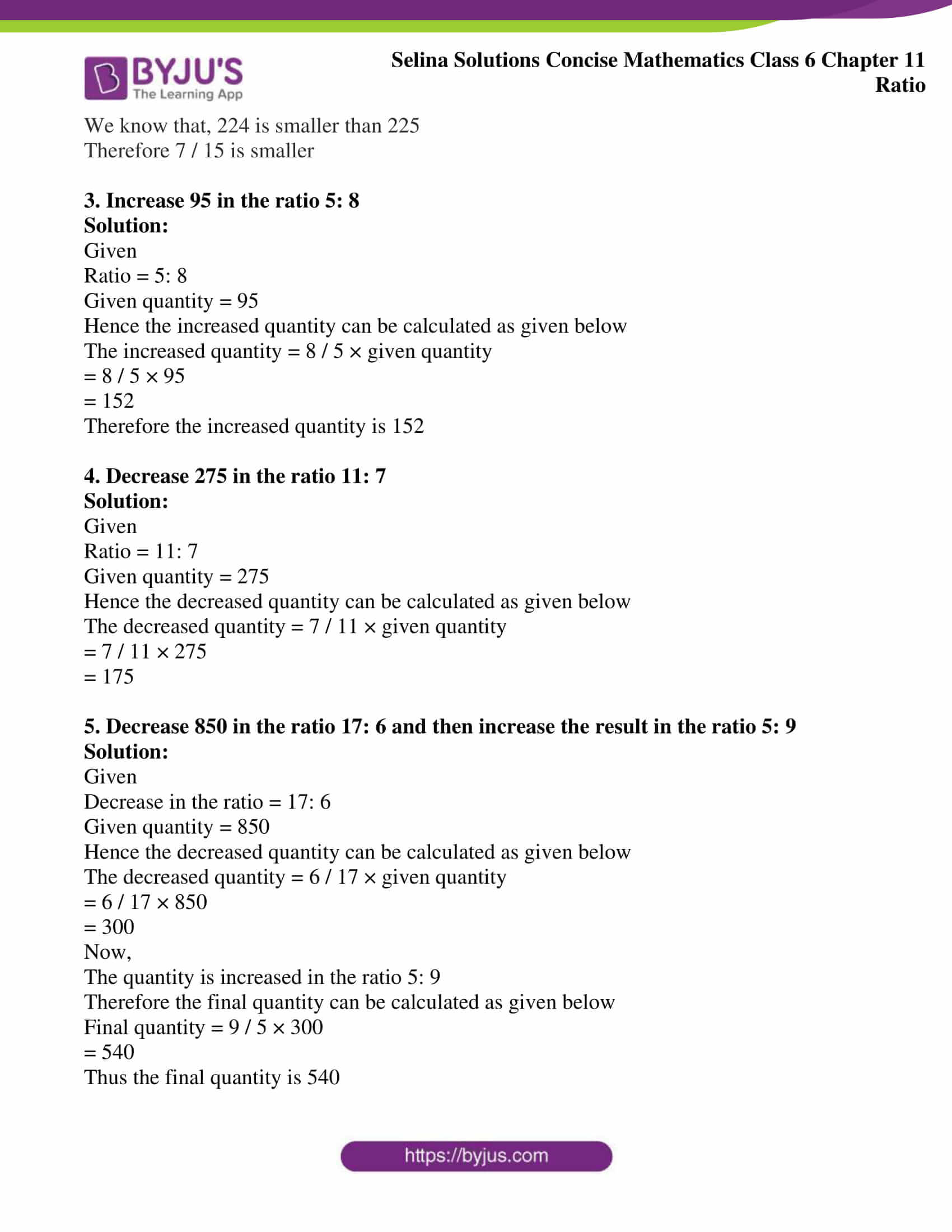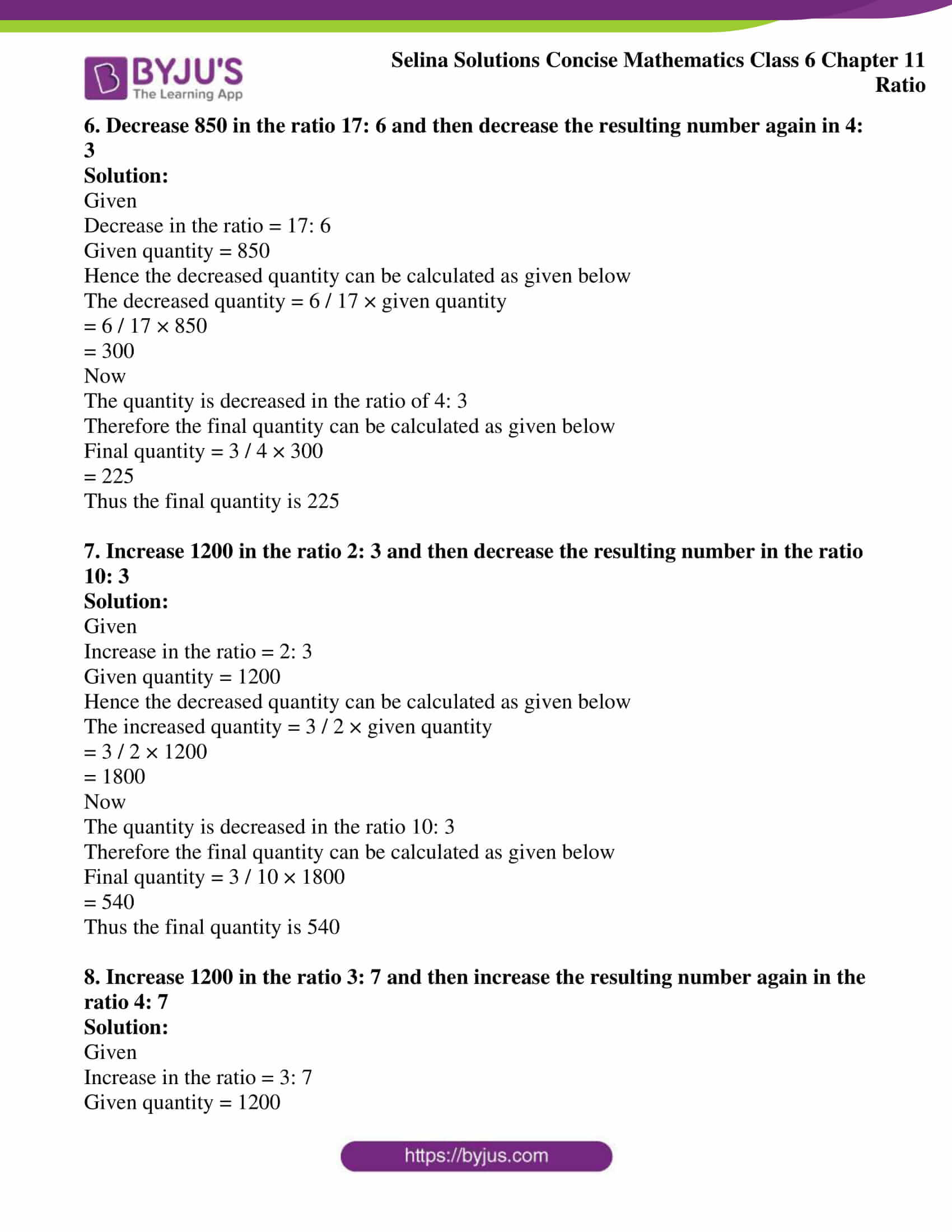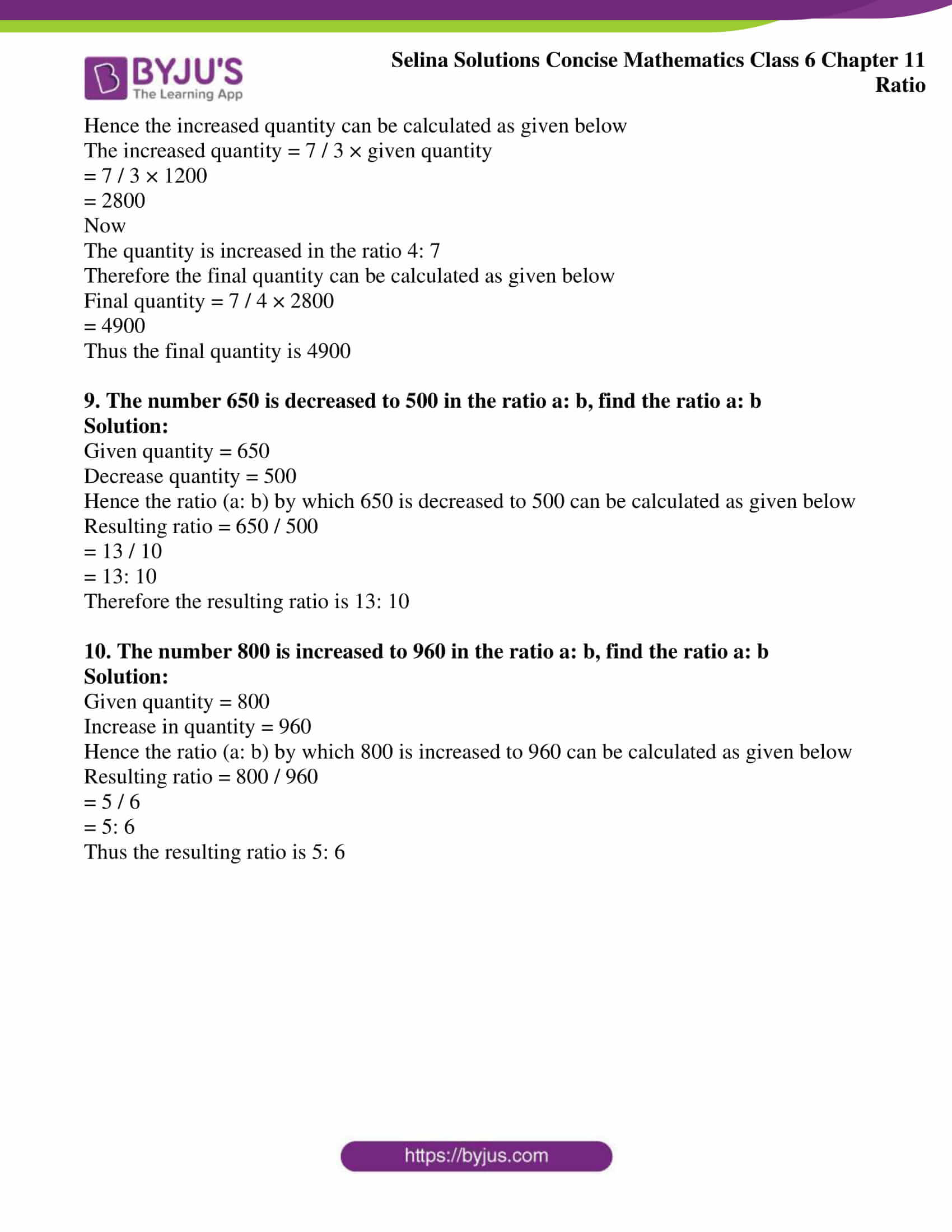## Access Selina Solutions Concise Mathematics Class 6 Chapter 11: Ratio

### Exercise 11(A)

1. Express each of the following ratios in its simplest form

(a) (i) 4: 6

(ii) 48: 54

(iii) 200: 250

(b) (i) 5 kg: 800 gm

(ii) 30 cm: 2 m

(iii) 3m: 90 cm

(iv) 2 years: 9 months

(v) 1 hour: 45 minutes

(c) (i)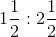(ii)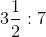(iii)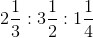(iv) x2: 4x

(v) 2.5: 1.5

Solution:

(a) (i) Given ratio

4: 6

This can be written as

4 / 6

= 2 / 3

= 2: 3

Hence, 2: 3 is the simplest form of 4: 6

(ii) Given

48: 54

This can be written as

48 / 54

= 8 / 9

= 8: 9

Hence, 8: 9 is the simplest form of 48: 54

(iii) Given

200: 250

This can be written as

200 / 250

= 4 / 5

= 4: 5

Hence, 4: 5 is the simplest form of 200: 250

(b) (i) Given

5 kg: 800 gm

5 kg = 5 × 1000 gm = 5000 gm

[∵ 1 kg = 1000 gm]

This can be written as

5000 gm / 800 gm

= 25 gm / 4 gm

= 25 gm: 4 gm

Hence, 25 gm: 4 gm is the simplest form of 5 kg: 800 gm

(ii) 30 cm: 2 m

We know that, 1 m = 100 cm

2 m = 2 × 100 cm

= 200 cm

Given

30 cm: 2 m

This can be written as

30 cm / 200 cm

= 3 cm / 20 cm

= 3 cm: 20 cm

Hence, 3 cm: 20 cm is the simplest form of 30 cm: 2 m

(iii) 3 m: 90 cm

We know that, 1 m = 100 cm

3 m = 3 × 100 cm

= 300 cm

Given

3 m: 90 cm

This can be written as

300 cm / 90 cm

= 10 cm / 3 cm

= 10 cm: 3 cm

Hence, 10 cm: 3 cm is the simplest form of 3 m: 90 cm

(iv) 2 years: 9 months

We know that, 1 year = 12 months

2 years = 2 × 12 months

= 24 months

Given

2 years: 9 months

This can be written as

24 months / 9 months

= 8 months / 3 months

= 8 months: 3 months

Hence, 8 months: 3 months is the simplest form of 2 years: 9 months

(v) 1 hour: 45 minutes

We know that, 1 hour = 60 minutes

Given

1 hour: 45 minutes

This can be written as

60 minutes / 45 minutes

= 4 minutes / 3 minutes

= 4 minutes: 3 minutes

Hence, 4 minutes: 3 minutes is the simplest form of 1 hour: 45 minutes

(c) (i)This can be written as

3 / 2: 5 / 2

By further calculation, we get

3 / 2 × 2 / 5

= 3 / 5

= 3: 5

Hence, the simplest form ofis 3: 5

(ii)This can be written as

7 / 2: 7 / 1

On further calculation, we get

7 / 2 × 1 / 7

= 1 / 2

= 1: 2

Hence, the simplest form ofis 1: 2

(iii)This can be written as

7 / 3: 7 / 2: 5 / 4

Now, taking L.C.M of 3, 2 and 4 we get

7 / 3 × 12: 7 / 2 × 12: 5 / 4 × 12

= 28: 42: 15

Hence, the simplest form ofis 28: 42: 15

(iv) x2: 4x

This can be written as

x2 / 4x

= (x × x) / (4 × x)

= x / 4

= x: 4

Hence, the simplest form of x2: 4x is x: 4

(v) 2.5: 1.5

This can be written as

25 / 10: 15 / 10

On further calculation, we get

= 25 / 10 × 10 / 15

= 25 / 15

= 5 / 3

= 5: 3

Hence, the simplest form of 2.5: 1.5 is 5: 3

2. A field is 80 m long and 60 m wide. Find the ratio of its width to its length.

Solution:

Given

Width of the field = 60 m

Length of the field = 80 m

Ratio of its width to its length = 60: 80

On further simplification, we get

= 60 / 80

= 3 / 4

= 3: 4

Hence, the ratio of its width to its length is 3: 4

3. State, true or false:

(i) A ratio equivalent to 7: 9 is 27: 21

(ii) A ratio equivalent to 5: 4 is 240: 192

(iii) A ratio of 250 gm and 3 kg is 1: 12

Solution:

(i) False

Correct statement: A ratio equivalent to 7: 9 is 9: 7

(ii) True

(iii) True

4. Is the ratio of 15 kg and 35 kg same as the ratio of 6 years and 14 years?

Solution:

Ratio of 15 kg and 35 kg = 15 kg: 35 kg

We get

15 kg / 35 kg

On simplification, we get

= 3 kg / 7 kg

= 3: 7

Now, the ratio of 6 years and 14 years = 6 years: 14 years

We get

6 years / 14 years

On simplification, we get

= 3 years / 7 years

= 3: 7

Since both the ratios = 3: 7

Hence, the ratios are same in both the cases

5. Is the ratio of 6 g and 15 g same as the ratio of 36 cm and 90 cm?

Solution:

Ratio of 6 g and 15 g = 6 g: 15 g

On calculating further, we get

= 6 / 15

= 2 / 5

= 2: 5

Now, the ratio of 36 cm and 90 cm = 36cm: 90 cm

By calculating further, we get

= 36 / 90

= 18 / 45

= 6 / 15

= 2 / 5

= 2: 5

Since both the ratios = 2: 5

Hence, the ratios are same in both the cases

6. Find the ratio between 3.5 m, 475 cm and 2.8 m

Solution:

Given

3.5 m, 475 cm and 2.8 m

Now, convert all the values into cm

1 m = 100 cm

Hence,

3.5 × 100 = 350 cm

2.8 × 100 = 280 cm

Hence,

350 cm: 475 cm: 280 cm

The H.C.F. of 350, 475 and 280 is 5

So, the ratio will be

350 / 5: 475 / 5: 280 / 5

= 70: 95: 56

Therefore, the ratio between 3.5 m, 475 cm and 2.8 m is 70: 95: 56

7. Find the ratio between 5 dozen and 2 scores. [1 score = 20]

Solution:

Given

5 dozens and 2 scores

We know that,

1 dozen = 12 and 1 score = 20

Hence,

5 dozens = 12 × 5

= 60

2 scores = 2 × 20

= 40

So, the ratio will be

60: 40 = 60 / 40

= 3 / 2

= 3: 2

Hence, the ratio between 5 dozens and 2 scores is 3: 2

### Exercise 11 (B)

1. The monthly salary of a person is Rs 12,000 and his monthly expenditure is Rs 8,500. Find the ratio of his:

(i) salary to expenditure

(ii) expenditure to savings

(iii) savings to salary

Solution:

Given

The monthly salary of a person = Rs 12, 000

Monthly expenditure = Rs 8, 500

(i) Salary to expenditure will be as given below

12, 000: 8, 500 = 12, 000 / 8, 500

On simplification, we get

= 120 / 85

= 24 / 17

= 24: 17

∴ The ratio between salary and expenditure is 24: 17

(ii) Savings = salary – expenditure

Savings = 12, 000 – 8, 500

= 3, 500

The ratio between expenditure and savings will be as given below

8500: 3500 = 8500 / 3500

On simplification, we get

= 85 / 35

= 17 / 7

= 17: 7

∴ The ratio between expenditure and savings will be 17: 7

(iii) Savings = salary – expenditure

Savings = 12, 000 – 8, 500

= 3, 500

The ratio between savings and salary will be as given below

3, 500: 12, 000 = 3500 / 12000

On simplification, we get

= 35 / 120

= 7 / 24

= 7: 24

∴ The ratio between savings and salary will be 7: 24

2. The strength of a class is 65, including 30 girls. Find the ratio of the number of:

(i) girls to boys

(ii) boys to the whole class

(iii) the whole class to girls

Solution:

Given

Total strength of class = 65

Total strength of girls = 30

Hence, total number of boys in a class will be

Boys = 65 – 30

= 35

(i) The ratio of girls to boys will be as given below:

30: 35 = 30 / 35

On calculating further, we get

= 6 / 7

= 6: 7

∴ The ratio between girls and boys will be 6: 7

(ii) Ratio of boys to the whole class will be as given below

35: 65 = 35 / 65

By calculating further, we get

= 7 / 13

= 7: 13

∴ The ratio between boys and whole class will be 7: 13

(iii) Ratio of whole class to the girls will be as given below

65: 30 = 65 / 30

On further calculation, we get

= 13 / 6

= 13: 6

∴ The ratio between whole class and girls will be 13: 6

3. The weekly expenses of a boy have increased from Rs 1, 500 to Rs 2, 250. Find the ratio of:

(i) increase in expenses to original expenses

(ii) original expenses to increased expenses

(iii) increased expenses to increase in expenses

Solution:

Given

Increased expenses of a boy = Rs 2, 250

Original expenses of a boy = Rs 1, 500

Hence, increase in expense will be:

Increase in expenses = 2250 – 1500

= 750

Hence, the ratio of increase in expenses to the original expenses will be:

750: 1500 = 750 / 1500

On calculation, we get

= 1 / 2

= 1: 2

∴ The ratio of increase in expenses to the original expenses will be 1: 2

(ii) The ratio of original expenses to increased expenses will be as given below

1500: 2250 = 1500 / 2250

On further calculation, we get

= 2 / 3

= 2: 3

∴ The ratio of original expenses to increased expenses will be 2: 3

(iii) The ratio of increased expenses to increase in expenses will be as given below

2250: 750 = 2250 / 750

On further calculation, we get

= 3 / 1

= 3: 1

∴ The ratio of increased expenses to increase in expenses will be 3: 1

4. Reduce each of the following ratios to their lowest terms:

(i) 1 hour 20 min: 2 hours

(ii) 4 weeks: 49 days

(iii) 3 years 4 months: 5 years 5 months

(iv) 2 m 40 cm: 1 m 44 cm

(v) 5 kg 500 gm: 2 kg 750 gm

Solution:

(i) 1 hour 20 min: 2 hours

We know that,

1 hour = 60 minutes

Hence, we can convert hour into minutes as:

1 hour = 1 × 60 minutes = 60 minutes

2 hours = 2 × 60 minutes = 120 minutes

So, the above expression can be written as follows:

(60 + 20) minutes / 120 minutes = 80 / 120

On further calculation, we get

= 2 / 3

= 2: 3

∴ The ratio of 1 hour 20 minutes: 2 hours will be 2: 3

(ii) 4 weeks: 49 days

We know that,

1 week = 7 days

Hence, we can convert weeks into days as given below

4 weeks = 4 × 7 days

= 28 days

So, the above expression can be written as follows:

28 days / 49 days = 4 / 7

We get

= 4: 7

∴ The ratio of 4 weeks: 49 days will be 4: 7

(iii) 3 years 4 months: 5 years 5 months

We know that,

1 year = 12 months

Hence, we can convert years into months as follows:

3 years = 3 × 12 months

= 36 months

5 years = 5 × 12 months

= 60 months

So, the above expression can be written as follows:

(36 + 4) months / (60 + 5) months = 40 / 65

On further calculation, we get

= 8 / 13

= 8: 13

∴ The ratio of 3 years 4 months: 5 years 5 months will be 8: 13

(iv) 2 m 40 cm: 1 m 44 cm

We know that,

1 metre = 100 cm

So, we can convert meter into centimetre as follows:

2 metre = 2 × 100 centimetres

= 200 centimetres

1 metre = 1 × 100 centimetre

= 100 centimetres

So, the above expression can be written as follows:

(200 + 40) centimetres / (100 + 44) centimetres = 240 / 144

On calculating further, we get

= 20 / 12

= 5 / 3

= 5: 3

∴ The ratio of 2 m 40 cm: 1 m 44 cm will be 5: 3

(v) 5 kg 500 gm: 2 kg 750 gm

We know that,

1 kilogram = 1000 gram

So, we can convert kilogram into gram as follows:

5 kilogram = 5 × 1000 gram

= 5000 gram

2 kilogram = 2 × 1000 gram

= 2000 gram

So, the above expression can be written as follows:

(5000 + 500) gram / (2000 + 750) gram = 5500 / 2750

On further calculation, we get

= 2 / 1

= 2: 1

∴ The ratio of 5 kg 500 gm: 2 kg 750 gm will be 2: 1

5. Two numbers are in the ratio 9: 2. If the smaller number is 320, find the larger number.

Solution:

Given

Two numbers are in the ratio = 9: 2

Smaller number = 320

Now, let us assume that the larger number is 9x and the smaller number is 2x

Therefore, the larger number = (9x × 320) / 2x

= 1440

Hence, the larger number = 1440

6. A bus travels 180 km in 3 hours and a train travels 450 km in 5 hours. Find the ratio of speed of train to speed of bus.

Solution:

Given

Total distance travelled by a bus = 180 km

Time taken by bus = 3 hours

Total distance travelled by train = 450 km

Time taken by train = 5 hours

We know that,

Speed = distance / time

Hence,

Speed of a bus = 180 km / 3 hr

= 60 km / hr

Speed of a train = 450 km / 5 hr

= 90 km / hr

Thus, ratio of speed of train to speed of bus will be

90: 60 = 90 / 60

We get

= 3: 2

7. In winters, a school opens at 10 a.m. and closes at 3.30 p.m. If the lunch interval is of 30 minutes, find the ratio of lunch interval to total time of the class periods.

Solution:

Given

School opens at = 10 a.m.

School closes at = 3.30 p.m.

Lunch interval timing of school = 30 minutes

Hence, total school timing will be 5 hours 30 minutes

Total time of class periods will be as follows:

Total time interval of class = Total school timings – lunch interval timing

= 5 hour 30 minutes – 30 minutes

= 5 hours

We know that,

1 hour = 60 minutes

So, we can convert hours into minutes as shown below

5 hour = 5 × 60 minutes

= 300 minutes

Thus, ratio of lunch interval to total class time will be

30 min: 300 min = 30 / 300

On calculation, we get

= 1 / 10

= 1: 10

∴ The ratio of lunch interval to total time of class periods will be 1: 10

8. Rohit goes to school by car at 60 km per hour and Manoj goes to school by scooty at 40 km per hour. If they both live in the same locality, find the ratio between the time taken by Rohit and Manoj to reach school.

Solution:

Given

Rohit car speed = 60 km/hr

Manoj car speed = 40 km/hr

Since, it is given that, they stay in the same locality

Hence, let the distance be x

We know

Time = Distance / Speed

Hence, time taken by Rohit to reach school will be:

Time taken by Rohit = x / 60

Time taken by Manoj = x / 40

Hence, ratio of time taken by Rohit and Manoj to reach school will be as follows:

x / 60: x / 40 = 1 / 3: 1 / 2

= 2 / 3

= 2: 3

Hence, the ratio between the time taken by Rohit and Manoj to reach school is 2: 3.

9. In a club having 360 members, 40 play carom, 96 play table tennis, 144 play badminton and remaining members play volley-ball. If no member plays two or more games, find the ratio of members who play:

(i) carom to the number of those who play badminton

(ii) badminton to the number of those who play table-tennis

(iii) table-tennis to the number of those who play volley-ball

(iv) volley-ball to the number of those who play other games

Solution:

Given

Total number of members in a club = 360 members

Total number of members who play carom = 40 members

Total number of members who play table tennis = 96 members

Total number of members who play badminton = 144 members

Hence, total number of members who play volley ball will be as follows:

360 – (40 + 96 + 144) = 360 – 280

= 80

(i) Hence, the ratio between the members who play carom to the number of those who play badminton will be:

40: 144 = 40 / 144

We get

= 5 / 18

= 5: 18

(ii) Hence, the ratio between the members who play badminton to the number of those who play table tennis will be:

144: 96 = 144 / 96

We get

= 6 / 4

= 3 / 2

= 3: 2

(iii) Hence, the ratio between the members who play table tennis to the number of those who play volley ball will be:

96: 80 = 96 / 80

We get

= 6 / 5

= 6: 5

(iv) Number of members who play other games than volley ball will be:

360 – 80 = 280

Hence, the ratio between the members who play volley ball to those members who play other games will be:

80: 280 = 80 / 280

On simplification, we get

= 4 / 14

= 2 / 7

= 2: 7

10. The length of a pencil is 18 cm and its radius is 4 cm. Find the ratio of its length to its diameter.

Solution:

Given

The length of a pencil = 18 cm

Radius of a pencil = 4 cm

We know that,

So,

Diameter of a pencil = 2 × 4

= 8 cm

Hence, ratio of pencil length to its diameter will be:

18: 8 = 18 / 8

We get

= 9 / 4

= 9: 4

11. Ratio of distance of the school from A’s home to the distance of the school from B’s home is 2: 1

(i) Who lives nearer to the school?

(ii) Complete the following table:

Solution:

(i) B lives nearer to school than A because

Since, it is given that, A’s home distance from school: B’s home distance from school = 2: 1

(A’s home distance from school) / (B’s home distance from school) = 2 / 1

Hence, A’s home distance from school = 2 × B’s home distance from school

(ii) Let A’s home is 2x km from school and B’s home is x km

Hence,

A’s home distance from school: B’s home distance from school = 2: 1

(A’s home distance from school) / (B’s home distance from school) = 2 / 1

A’s home distance from school = 2 × B’s home distance from school

(a) So if A lives at a distance of 4 km then B will live at a distance of = 1 / 2 × 4

= 2 km

(b) So if B lives at a distance of 9 km then A will live at a distance of = 2 × 9

= 18 km

(c) So if A lives at a distance of 8 km then B will live at a distance of = 1 / 2 × 8

= 4 km

(d) So if B lives at a distance of 8 km the n A will live at a distance of = 2 × 8

= 16 km

(e) So if A lives at a distance of 6 km then B will live at a distance of = 1 / 2 × 6

= 3 km

12. The student-teacher ratio in a school is 45: 2. If there are 4050 students in the school, how many teachers must be there?

Solution:

Given

Total number of students in school = 4050

Student –teacher ratio in a school = 45: 2

Let us assume that the total number of teachers in school be x

Hence,

Required ratio = Total number of students / Total number of teachers

We get

45: 2 = 4050: x

45 / 2 = 4050 / x

x = (4050 × 2) / 45

x = 8100 / 45

x = 180 teachers

### Exercise 11(C)

1. Rs 120 is to be divided between Hari and Gopi in the ratio 5: 3. How much does each get?

Solution:

Given

Total amount = Rs 120

Amount divided between Hari and Gopi in the ratio = 5: 3

Hence,

The sum of ratio is as follows:

Sum of ratio = 5 + 3

= 8

Hence, Hari’s and Gopi’s share will be as follows:

Hari’s share = (120 × 5) / 8

= Rs 75

Gopi’s share = (120 × 3) / 8

= Rs 45

Therefore, Hari get Rs 75 and Gopi get Rs 45

2. Divide 72 in the ratio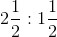Solution:

Given

Ratio =Number = 72

The above expression can be written as follows:= 5 / 2: 3 / 2

We get

= 5 / 2 × 2: 3 / 2 × 2

= 5: 3

Thus, the sum of ratios is as follows:

Sum of ratio = 5 + 3

= 8

First divide = 5 / 8 × 72

= 45

Second divide = 3 / 8 × 72

= 27

3. Divide 81 into three parts in the ratio 2: 3: 4

Solution:

Given

Number = 81

Ratio = 2: 3: 4

Hence, the sum of ratio is calculated as follows

Sum of ratios = 2 + 3 + 4

= 9

First divide = 2 / 9 × 81

= 18

Second divide = 3 / 9 × 81

= 27

Third divide = 4 / 9 × 81

= 36

Therefore, 81 can be divided into 18, 27 and 36 in the ratio 2: 3: 4

4. Divide Rs 10, 400 among A, B and C in the ratio 1/ 2: 1/ 3: 1/ 4

Solution:

Given

Amount = Rs 10400

Amount to be divided into ratio = 1/ 2: 1/ 3: 1/ 4

The L.C.M of 2, 3 and 4 is 12

Hence, given ratio will be as given below

1 / 2: 1 / 3: 1 / 4 = 1 / 2 × 12: 1 / 3 × 12: 1 / 4 × 12

= 6: 4: 3

A’s part = 6 / 13 × 10400

We get

= 6 × 800

= 4800

B’s part = 4 / 13 × 10400

We get

= 4 × 800

= 3200

C’s part = 3 / 13 × 10400

We get

= 3 × 800

= 2400

5. A profit of Rs 2, 500 is to be shared among three persons in the ratio 6: 9: 10. How much does each person get?

Solution:

Given

Total profit = Rs 2, 500

Ratio = 6: 9: 10

Sum of ratio = 6 + 9 + 10

= 25

Share of first person = 6 / 25 × 2500

= 6 × 100

= 600

Share of second person = 9 / 25 × 2500

= 9 × 100

= 900

Share of third person = 10 / 25 × 2500

= 10 × 100

= 1000

6. The angles of a triangle are in the ratio 3: 7: 8. Find the greatest and the smallest angles.

Solution:

Given

Ratio = 3: 7: 8

We know that

Sum of angles of a triangle = 1800

Sum of ratios = 3 + 7 + 8

= 18

Hence, the angles are calculated as

Smallest angle = 3 / 18 × 1800

= 3 × 10

= 300

Greatest angle = 8 / 18 × 1800

= 8 × 10

= 800

7. The sides of a triangle are in the ratio 3: 2: 4. If the perimeter of the triangle is 27 cm, find the length of each side.

Solution:

Given

Ratio of the sides of a triangle is 3: 2: 4

Sum of ratios = 3 + 2 + 4

= 9

Perimeter of a triangle = 27 cm

Length of first side = 27 / 9 × 3

= 3 × 3

= 9 cm

Length of second side = 27 / 9 × 2

= 3 × 2

= 6 cm

Length of third side = 27 / 9 × 4

= 3 × 4

= 12 cm

8. An alloy of zinc and copper weighs 12 ½ kg. If in the alloy, the ratio of zinc and copper is 1: 4, find the weight of copper in it.

Solution:

Given

Weight of an alloy = 12 ½ kg

= 25 / 2 kg

Ratio of zinc and copper = 1:4

Sum of ratios = 1 + 4

= 5

Hence, weight of copper will be as given below

Weight of copper = 4 / 5 × 25 / 2 kg

= 2 × 5

= 10 kg

Therefore, weight of copper in it is 10 kg

9. How will Rs 31500 be shared between A, B and C; if A gets the double of what B gets, and B gets the double of what C gets?

Solution:

Given

Amount = Rs 31500

Let the share of C = 1

Share of B = double of C

= 2 × 1

= 2

Share of A = double of B

= 2 × 2

= 4

Therefore, given ratio will be

A: B: C = 4: 2: 1

Sum of ratios = 4 + 2 + 1

= 7

A’s share = 4 / 7 × 31500

= 4 × 4500

= Rs 18000

B’s share = 2 / 7 × 31500

= 2 × 4500

= Rs 9000

C’s share = 1 / 7 × 31500

= 1 × 4500

= Rs 4500

10. Mr. Gupta divides Rs 81000 among his three children Ashok, Mohit and Geeta in such a way that Ashok gets four times what Mohit gets and Mohit gets 2.5 times what Geeta gets. Find the share of each of them.

Solution:

Given

Amount = Rs 81000

Let the share of Geeta = 1

Share of Mohit is 2.5 times of Geeta

Hence, share of Mohit becomes = 2.5

Share of Ashok is 4 times of Mohit

Hence, share of Ashok becomes = 4 × 2.5

= 10

Ratio = 1: 2.5: 10

= 1 × 2: 2.5 × 2: 10 × 2

= 2: 5: 20

Thus, sum of ratios = 2 + 5 + 20

= 27

Share of Geeta = 2 / 27 × 81000

= 2 × 3000

= Rs 6000

Share of Mohit = 5 / 27 × 81000

= 5 × 3000

= Rs 15000

Share of Ashok = 20 / 27 × 81000

= 20 × 3000

= Rs 60000

### Exercise 11(D)

1. Which ratio is greater:

(i) 8 / 15 or 5 / 9

(ii) 3 / 7 or 6 / 13

Solution:

(i) Given

8 / 15 or 5 / 9

The above expression can be written as follows:

8 / 15 or 5 / 9

= 8 × 9 or 15 × 5

= 72 or 75

We know that 75 is greater than 72

Therefore 5 / 9 is greater

(ii) 3 / 7 or 6 / 13

The above expression can be written as follows:

3 / 7 or 6 / 13

⇒ 3 × 13 or 6 × 7

⇒ 39 or 42

We know that 42 is greater than 39

Therefore 6 / 13 is greater

2. Which ratio is smaller:

(i) 9 / 17 or 8 / 15

(ii) 7 / 15 or 15 / 32

Solution:

(i) Given

9 / 17 or 8 / 15

The above expression can be written as follows:

9 / 17 or 8 / 15

⇒ 9 × 15 or 8 × 17

⇒ 135 or 136

We know that, 135 is smaller than 136

Therefore 9 / 17 is smaller

(ii) Given

7 / 15 or 15 / 32

The above expression can be written as follows:

7 / 15 or 15 / 32

⇒ 7 × 32 or 15 × 15

⇒ 224 or 225

We know that, 224 is smaller than 225

Therefore 7 / 15 is smaller

3. Increase 95 in the ratio 5: 8

Solution:

Given

Ratio = 5: 8

Given quantity = 95

Hence the increased quantity can be calculated as given below

The increased quantity = 8 / 5 × given quantity

= 8 / 5 × 95

= 152

Therefore the increased quantity is 152

4. Decrease 275 in the ratio 11: 7

Solution:

Given

Ratio = 11: 7

Given quantity = 275

Hence the decreased quantity can be calculated as given below

The decreased quantity = 7 / 11 × given quantity

= 7 / 11 × 275

= 175

5. Decrease 850 in the ratio 17: 6 and then increase the result in the ratio 5: 9

Solution:

Given

Decrease in the ratio = 17: 6

Given quantity = 850

Hence the decreased quantity can be calculated as given below

The decreased quantity = 6 / 17 × given quantity

= 6 / 17 × 850

= 300

Now,

The quantity is increased in the ratio 5: 9

Therefore the final quantity can be calculated as given below

Final quantity = 9 / 5 × 300

= 540

Thus the final quantity is 540

6. Decrease 850 in the ratio 17: 6 and then decrease the resulting number again in 4: 3

Solution:

Given

Decrease in the ratio = 17: 6

Given quantity = 850

Hence the decreased quantity can be calculated as given below

The decreased quantity = 6 / 17 × given quantity

= 6 / 17 × 850

= 300

Now

The quantity is decreased in the ratio of 4: 3

Therefore the final quantity can be calculated as given below

Final quantity = 3 / 4 × 300

= 225

Thus the final quantity is 225

7. Increase 1200 in the ratio 2: 3 and then decrease the resulting number in the ratio 10: 3

Solution:

Given

Increase in the ratio = 2: 3

Given quantity = 1200

Hence the decreased quantity can be calculated as given below

The increased quantity = 3 / 2 × given quantity

= 3 / 2 × 1200

= 1800

Now

The quantity is decreased in the ratio 10: 3

Therefore the final quantity can be calculated as given below

Final quantity = 3 / 10 × 1800

= 540

Thus the final quantity is 540

8. Increase 1200 in the ratio 3: 7 and then increase the resulting number again in the ratio 4: 7

Solution:

Given

Increase in the ratio = 3: 7

Given quantity = 1200

Hence the increased quantity can be calculated as given below

The increased quantity = 7 / 3 × given quantity

= 7 / 3 × 1200

= 2800

Now

The quantity is increased in the ratio 4: 7

Therefore the final quantity can be calculated as given below

Final quantity = 7 / 4 × 2800

= 4900

Thus the final quantity is 4900

9. The number 650 is decreased to 500 in the ratio a: b, find the ratio a: b

Solution:

Given quantity = 650

Decrease quantity = 500

Hence the ratio (a: b) by which 650 is decreased to 500 can be calculated as given below

Resulting ratio = 650 / 500

= 13 / 10

= 13: 10

Therefore the resulting ratio is 13: 10

10. The number 800 is increased to 960 in the ratio a: b, find the ratio a: b

Solution:

Given quantity = 800

Increase in quantity = 960

Hence the ratio (a: b) by which 800 is increased to 960 can be calculated as given below

Resulting ratio = 800 / 960

= 5 / 6

= 5: 6

Thus the resulting ratio is 5: 6

### Exercises of Selina Solutions Concise Mathematics Class 6 Chapter 11: Ratio

Exercise 11(A) Solutions

Exercise 11(B) Solutions

Exercise 11(C) Solutions

Exercise 11(D) Solutions